• 复变函数可以有多个值
千次阅读
2017-11-26 22:30:48

# 3 复变函数的积分

## 3.1 概念

闭曲线积分 Cf(z)dz=Cf(z)dz ∮ C f ( z ) d z = − ∮ C − f ( z ) d z $\oint\limits_{C}f(z)dz=-\oint\limits_{C^-}f(z)dz$

Cf(z)dz=Cudxvdy+iCvdx+udy ∮ C f ( z ) d z = ∫ C u d x − v d y + i ∫ C v d x + u d y $\oint\limits_{C}f(z)dz=\int\limits_{C}udx-vdy+i\int\limits_{C}vdx+udy$

Cf(z)dz=βαf[z(t)]z(t)dt ∮ C f ( z ) d z = ∫ α β f [ z ( t ) ] z ′ ( t ) d t $\oint\limits_{C}f(z)dz=\int_\alpha^\beta f[z(t)]z'(t)dt$

|zz0|=rdz(zz0)n+1={2πi,0,n=0n0 ∮ | z − z 0 | = r d z ( z − z 0 ) n + 1 = { 2 π i , n = 0 0 , n ≠ 0

估值不等式：曲线C长度为L，函数f(z)在C上满足|f(z)| $\le$M,则

|Cf(z)dz|C|f(z)|dsML | ∫ C f ( z ) d z | ≤ ∫ C | f ( z ) | d s ≤ M L $|\int\limits_{C}f(z)dz|\le \int\limits_{C}|f(z)|ds\le ML$

## 3.2 柯西-古萨基本定理

积分与路线无关。

柯西-古萨基本定理：如果f(z)在单连通域B内处处解析，那么f(z)在B内的任何一条封闭曲线C的积分为0。

Cf(z)dz=0 ∮ C f ( z ) d z = 0 $\oint\limits_{C}f(z)dz=0$

## 3.3 复合闭路定理

闭路变形原理：区域内一个解析函数沿闭曲线的积分，不因闭曲线在区域内作连续变形而改变它的值，只要变形过程中不经过不解析的点。

复合闭路定理：C为多连通域D内的一条简单闭曲线， C1,C2... C 1 , C 2 . . . $C_1,C_2...$ 为C内部的简单闭曲线，塔门互不包含互不相交，以它们为边界的区域全含于D，如果f(z)在D内解析，则

Cf(z)dz=k=1nCkf(z)dz ∮ C f ( z ) d z = ∑ k = 1 n ∮ C k f ( z ) d z $\oint\limits_{C}f(z)dz=\sum\limits_{k=1}^n\oint_{C_k}f(z)dz$

## 3.4 原函数与不定积分

如果函数在单连通区域内处处解析，那么积分与路线无关。

如果f(z)在单连通域B内处处解析，那么函数F(z)必为B内的解析函数。

## 3.5 柯西积分公式

f(z0)=12πiCf(z)zz0dz f ( z 0 ) = 1 2 π i ∮ C f ( z ) z − z 0 d z

如果C是圆周 z=z0+Reiθ z = z 0 + R e i θ $z=z_0+Re^{i\theta}$,那么

f(z0)=12π2π0f(z0+Reiθ)dθ f ( z 0 ) = 1 2 π ∫ 0 2 π f ( z 0 + R e i θ ) d θ

即，一个解析函数在圆心处的值等于它在圆周上的平均值。

逆定理：魔勒拉

## 3.6 解析函数的高阶导数

f(n)(z0)=n!2πiCf(z)(zz0)n+1dz f ( n ) ( z 0 ) = n ! 2 π i ∮ C f ( z ) ( z − z 0 ) n + 1 d z

## 3.7 调和函数

如果二元实变函数 φ(x,y) φ ( x , y ) $\varphi(x,y)$在区域D内具有二阶连续偏导数并且满足拉普拉斯方程

2φx2+2φy2=0 ∂ 2 φ ∂ x 2 + ∂ 2 φ ∂ y 2 = 0

那么称 φ(x,y) φ ( x , y ) $\varphi(x,y)$为D内的调和函数。

D内的解析函数的虚部为实部的共轭调和函数。

更多相关内容
• 复变函数部分容包括复变函数及其导数和积分、复变函数的级数、留数及其应用；数理方程部分包括数学物理方程及其定解条件、分离变量法、 Sturm-Liouville（施图姆-刘维尔）本征问题、贝塞尔函数及其应用、行波法与...
• 复变函数（第五版）/面向21世纪课程教材》内容包括：复数及复平面、复变函数复变函数的积分、级数、留数、保形映射、解析开拓以及调和函数共八章，其中除单性定理外，均属于复变函数课程的一般内容。...
• 编写本书的目标是将复变函数的教学放在一更大的整体框架中考虑。在纵向上要配合后继教学以及学生从事科研的基础知识的需要，在横向上希望契合其他学科特别是理论物理学科的需求。实践证明，本教材较适合数学学院各...
• 利用复方法在,研究了多复变中含两复变量二阶方程的Riemann边问题,并给出了该问题的可解条件和通解的积分表达式,并进行了推广。
• 复变函数札记 出版时间：2011年版 内容简介 　《复变函数札记》是作者梁昌洪继《矢算场论札记》（科学出版社，2007）之后的第二本工程数学札记。尽管两书所涉及领域完全不同，但却有着完全一致的目标，即希望在数学...
• 具体由以下七部分组成：复数与复变函数；解析函数；复变函数的积分；解析函数的幂级数表示法；解析函数的洛朗展式与孤立奇点；留数理论及其应用；共形映射。 本课程的教学重点是：柯西—黎曼方程；柯西积分定理...
• 数理方法中复变函数、解析函数章节个人学习笔记，整理了复变函数及解析函数相关性质及应用，适合初学者快速学习了解，也可作复习资料翻阅复习。
• ## 复变函数基础概述

千次阅读 多人点赞 2019-08-07 20:06:31
由于一些原因没有参加学校的期末考试，也错过了学校的卓越班选拔（本来很把握的…）学校的一些课程也没有学完，所以只好在暑假卑微的看复变函数，看了一些下载的网课基本上有点感觉，做总结，之后的学习中应该也...

由于一些原因没有参加学校的期末考试，学校的一些课程也没有学完，所以只好在暑假卑微的看复变函数，看了一些下载的网课基本上有点感觉，做个总结，之后的学习中应该也那用到叭。

# 一.复数及其运算

## 1.求复数的虚部和实部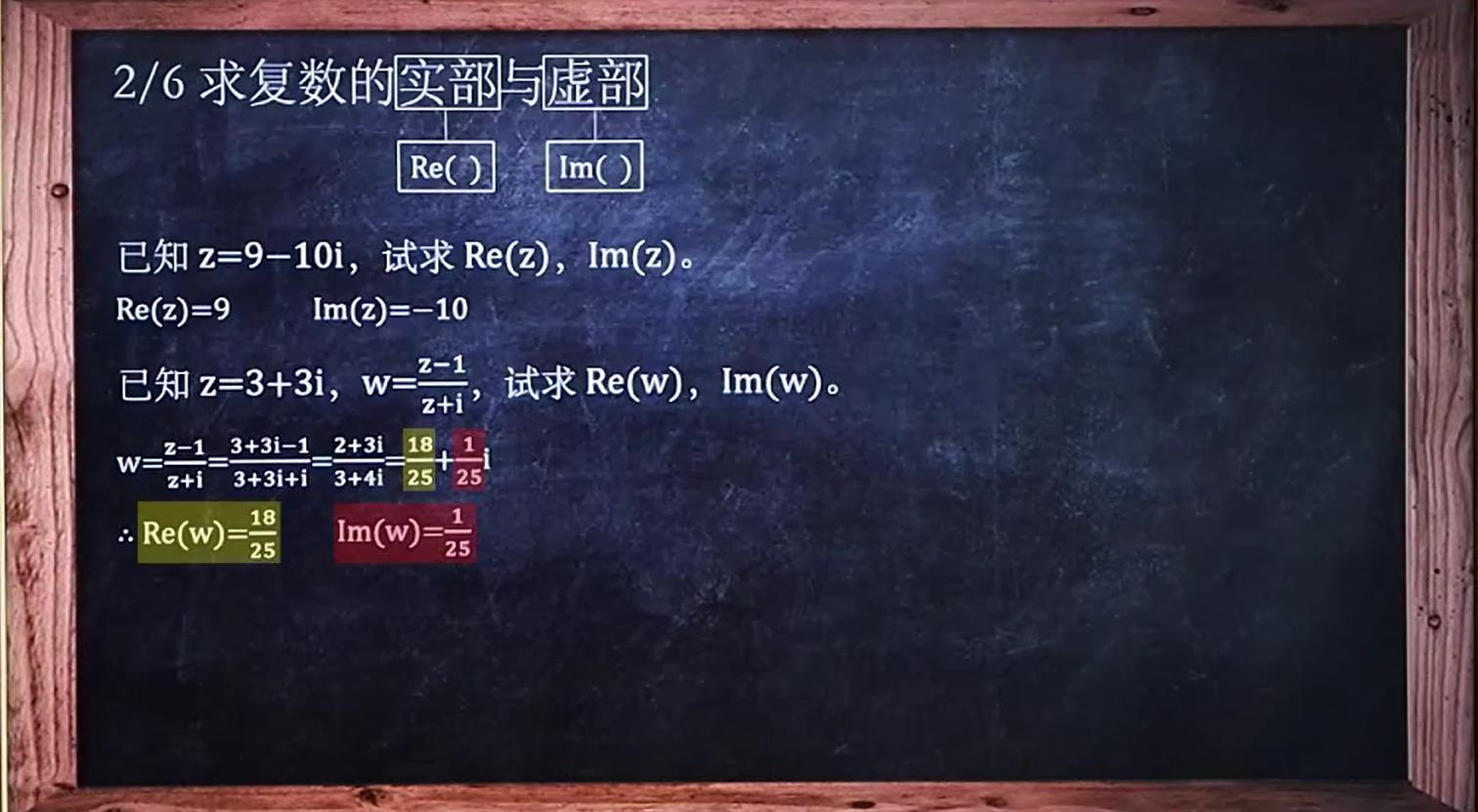只要把复数写出来，这种题目就迎刃而解吧。

## 2.求模、辐角和辐角主值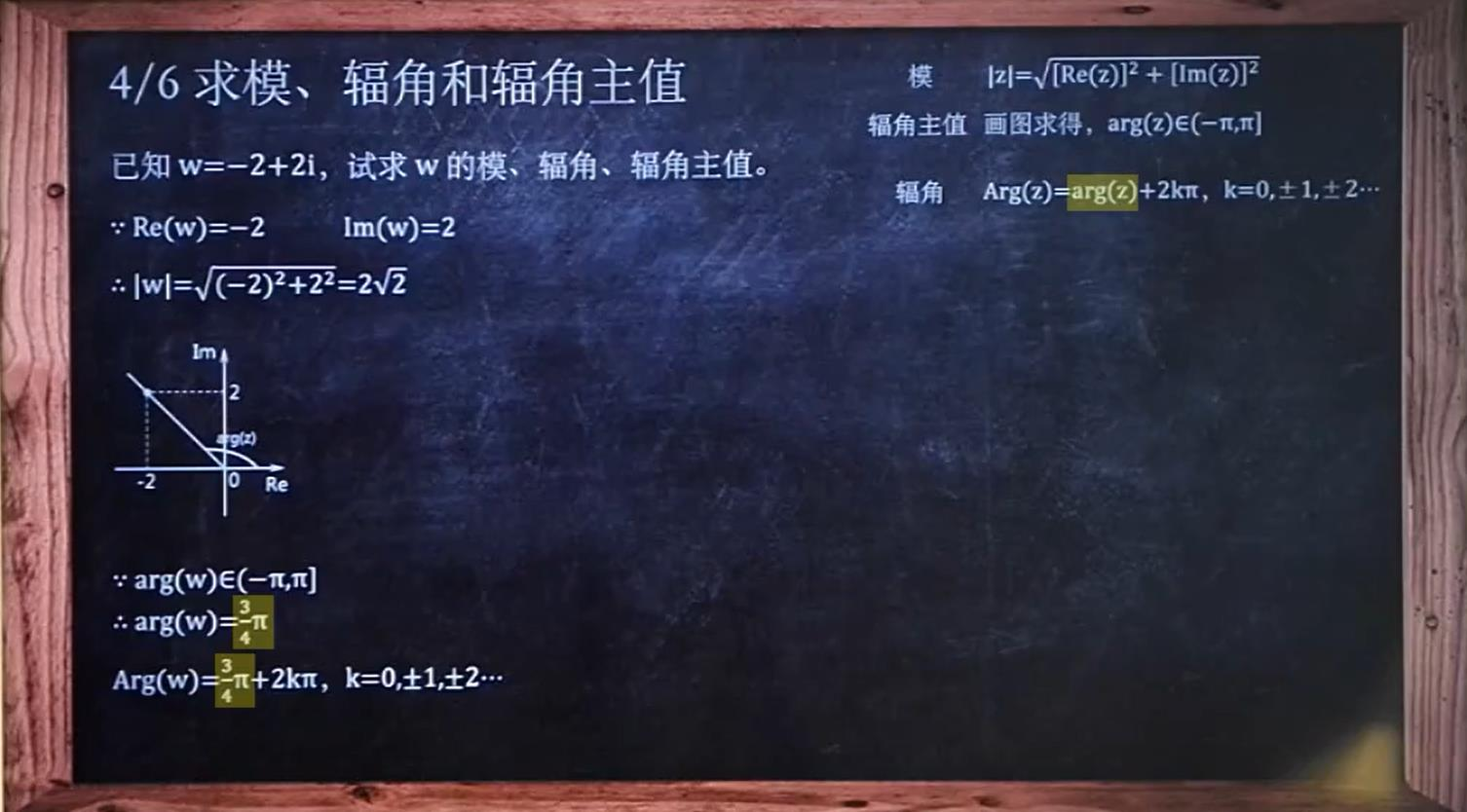求模：复数的求模和向量类似，把实部虚部平方和开方就行。
辐角主值：做出复数的图，辐角就是复数图像与实轴正方向的夹角，但其也有范围限制，要求在正负pi之间，是一个固定的值。
辐角：在辐角的基础上加周期，一个周期为2pi
另外还有一些基础的运算关系：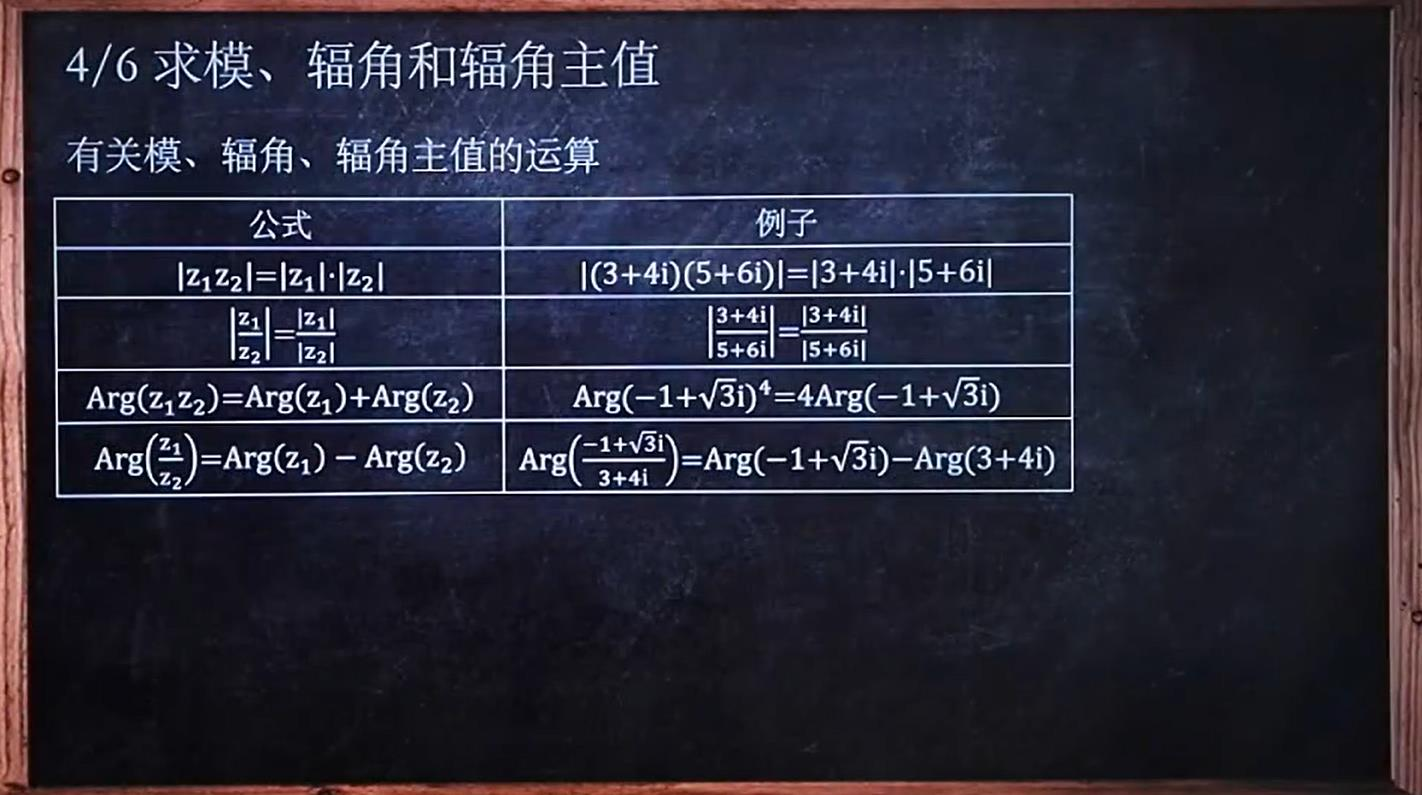## 3.复数开方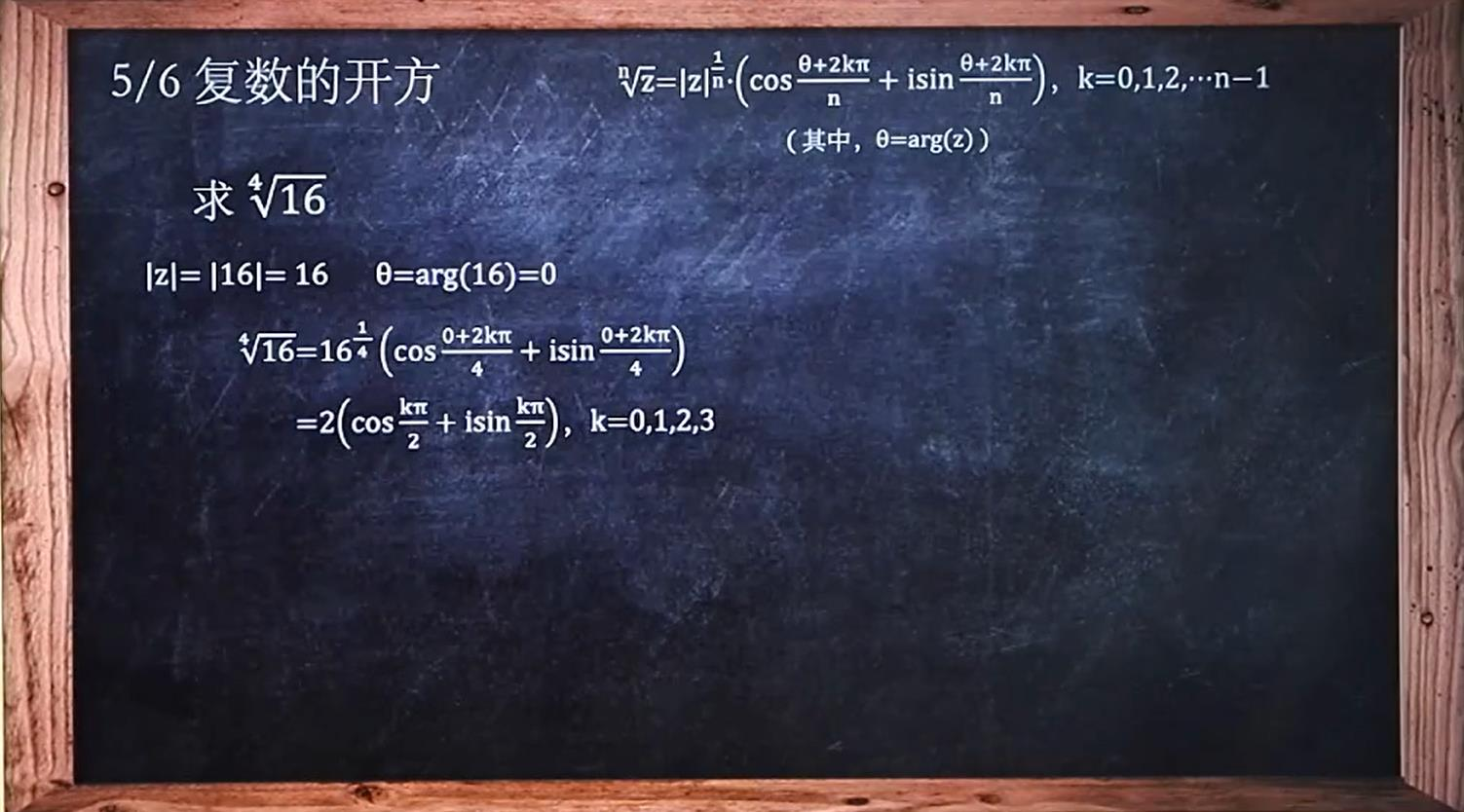复数的开方实际上是复数的幂运算的一种简单形式，有固定的套路，按套路带入相应的模和辐角主值即可。注意的是复数开n次方的就有n个结果，这与其图像对应，复平面上一个复数开n次根的图像就是以此复数的模为半径的一个圆的n分线。

## 4.代数式、三角式、指数式相互转换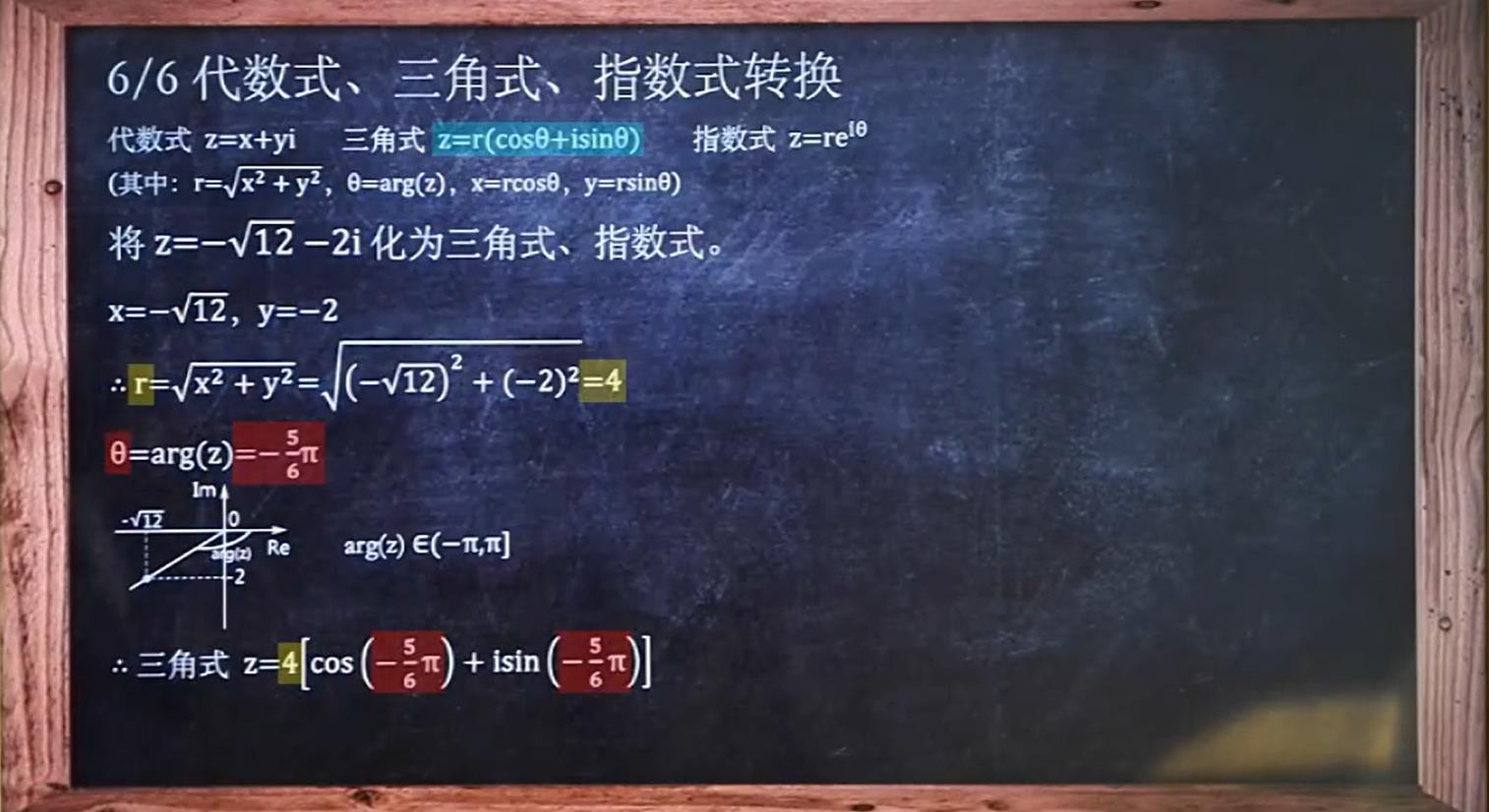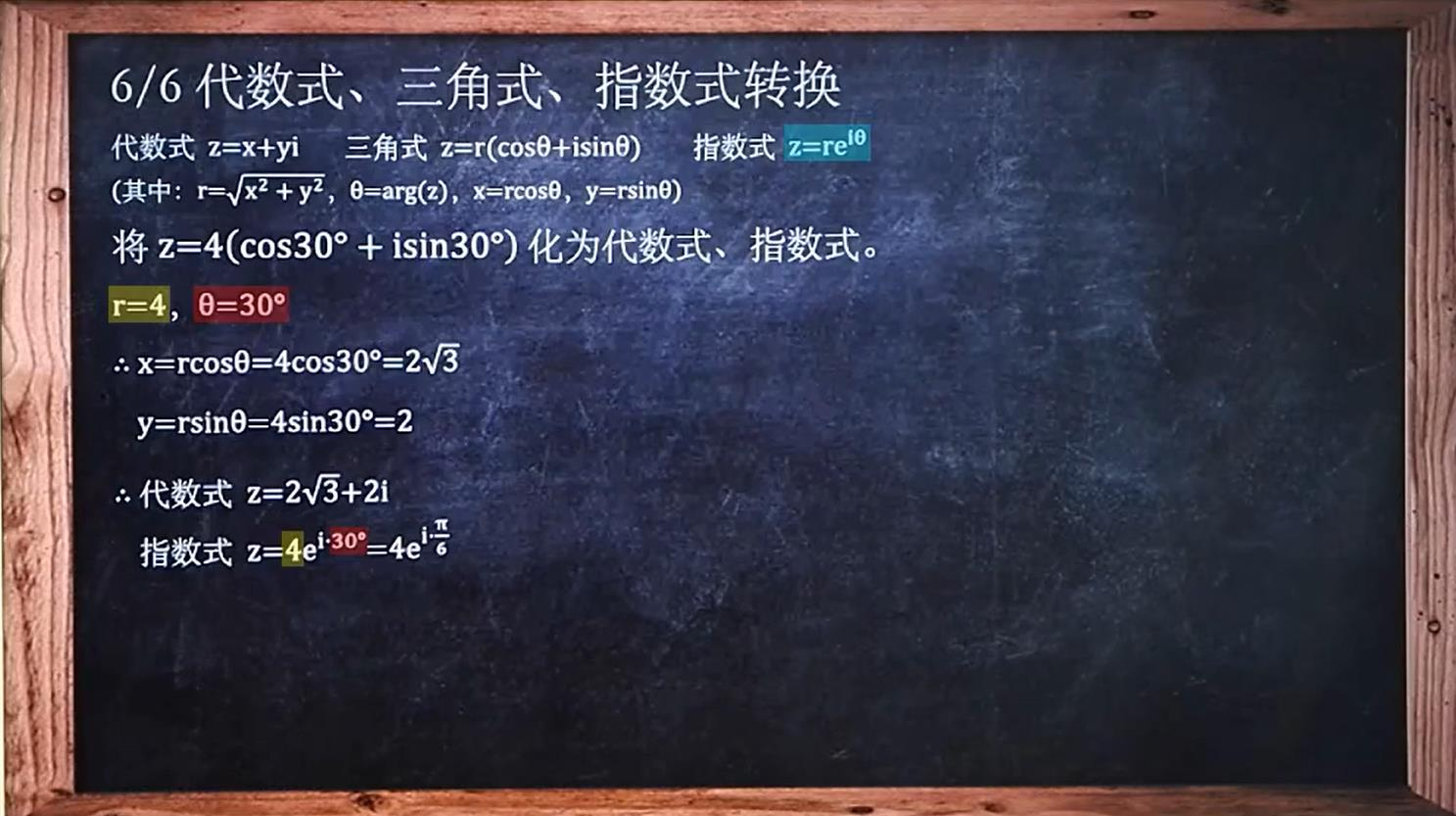三者之间的转换始终围绕着俩个因素：模和辐角主值，把握住这俩个灵魂，涉及这三者的转换问题就小菜一碟。
在后期的运算中经常需要对复数进行三个形态之间的转换。

# 二.复数形式的方程映射

前面一章节主要是针对复数本身的运算，这一章提升到了函数的层次，与直角坐标系建立连接，相当于是复变函数新知识和之前所学的函数的呼应吧。主要内容为函数的形式转换和映射。

## 1.将直角坐标方程化为复数形式方程

主要是直角坐标系下的一般方程和参数方程转换为复数形式。
参数方程：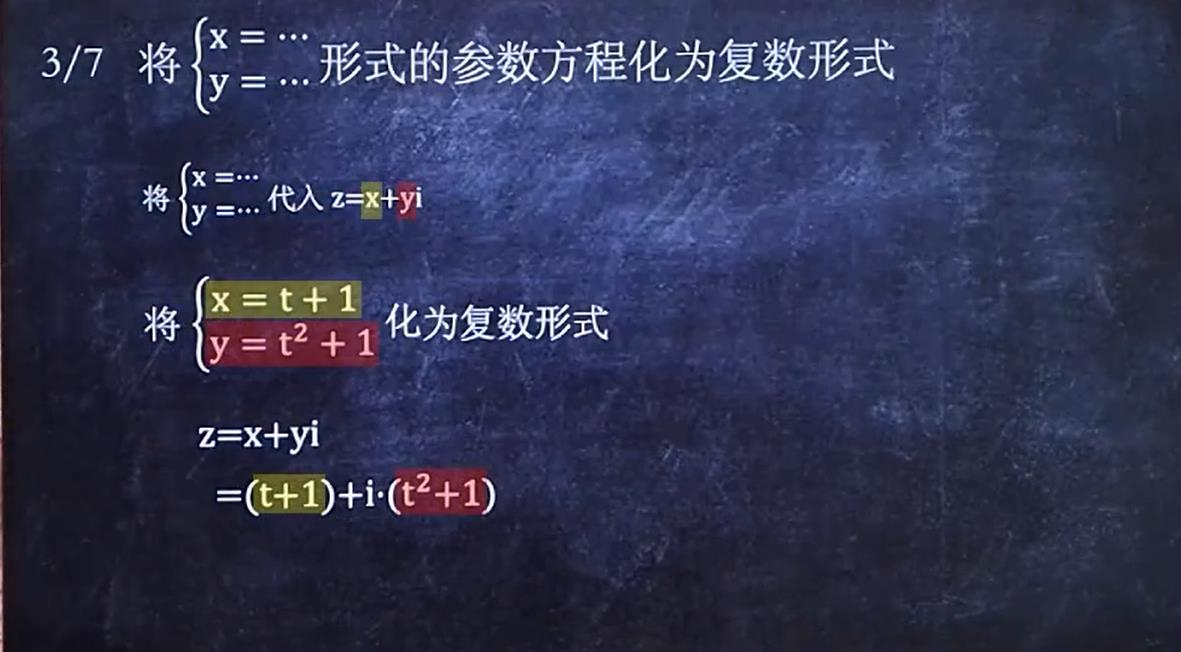参数方程的转换很简单，只要将xy原封不动的带入复数形式就行。
一般方程：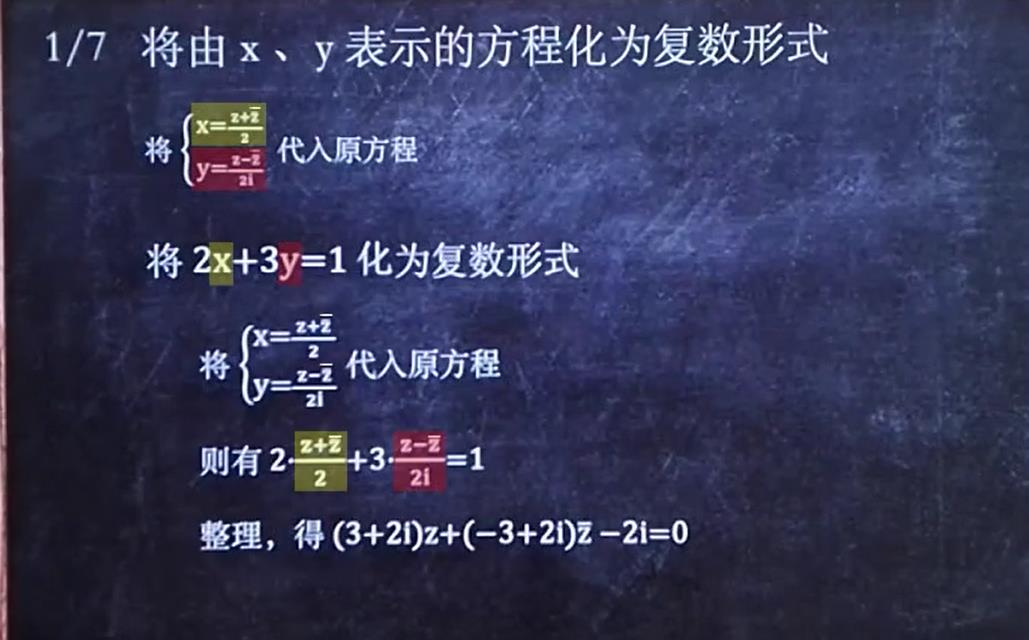一般方程的转换，宗旨是用复数替代x、y，所以解题方法就反向下手，将x、y用复数表示出然后带入原方程就得到了复数形式。利用复数与其共轭复数相加的运算可得出x、y与复数的关系。

## 2.将复数形式方程转换为直角坐标方程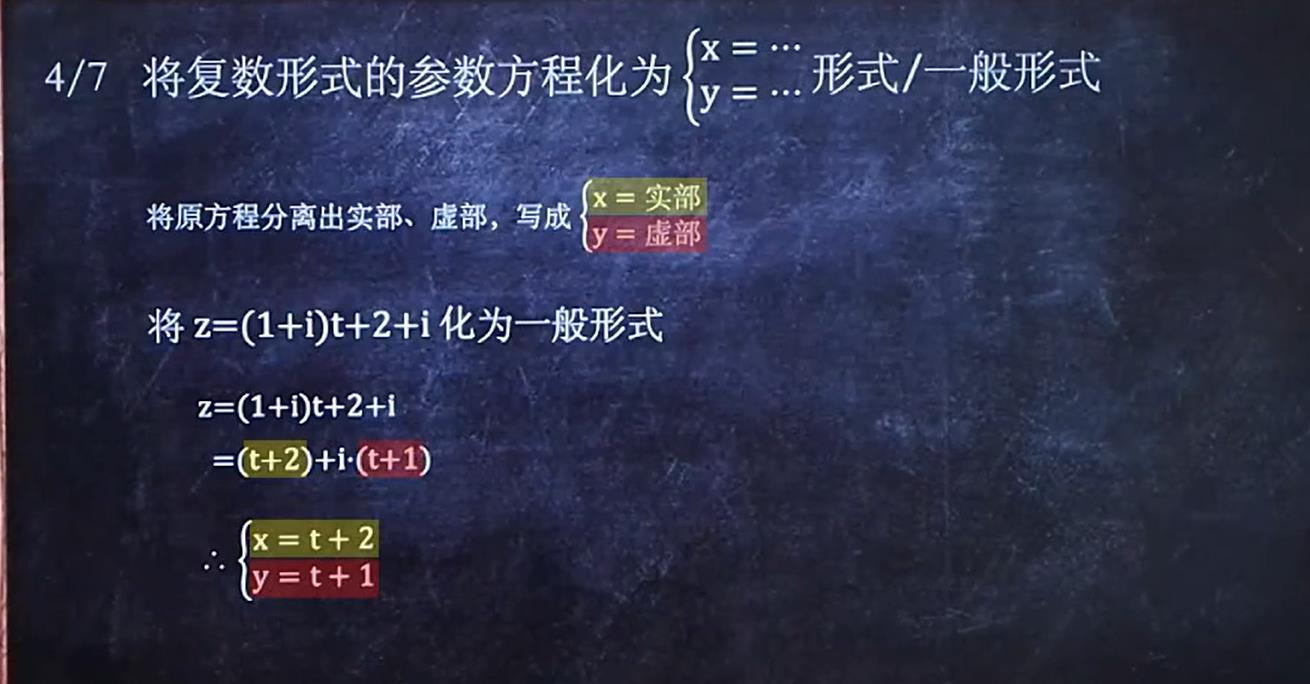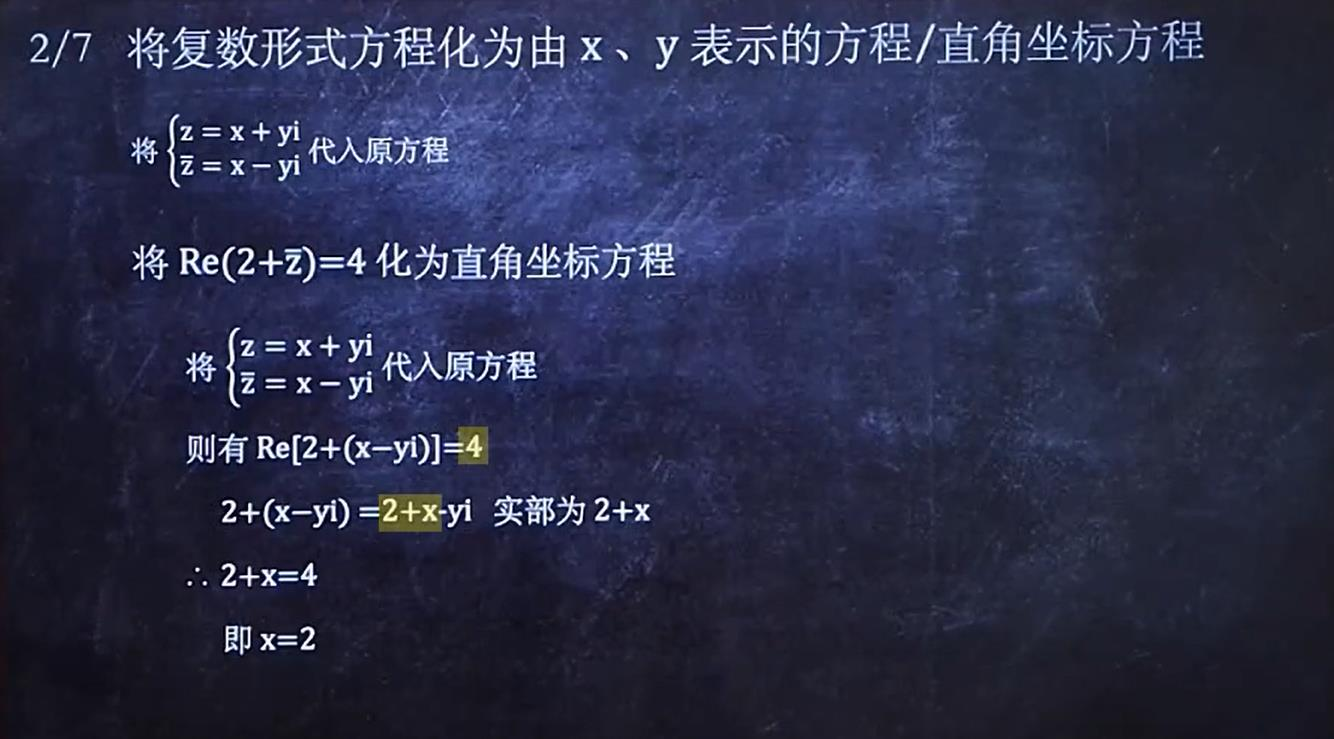解题方法与上一节相反。

## 3.求映射下的象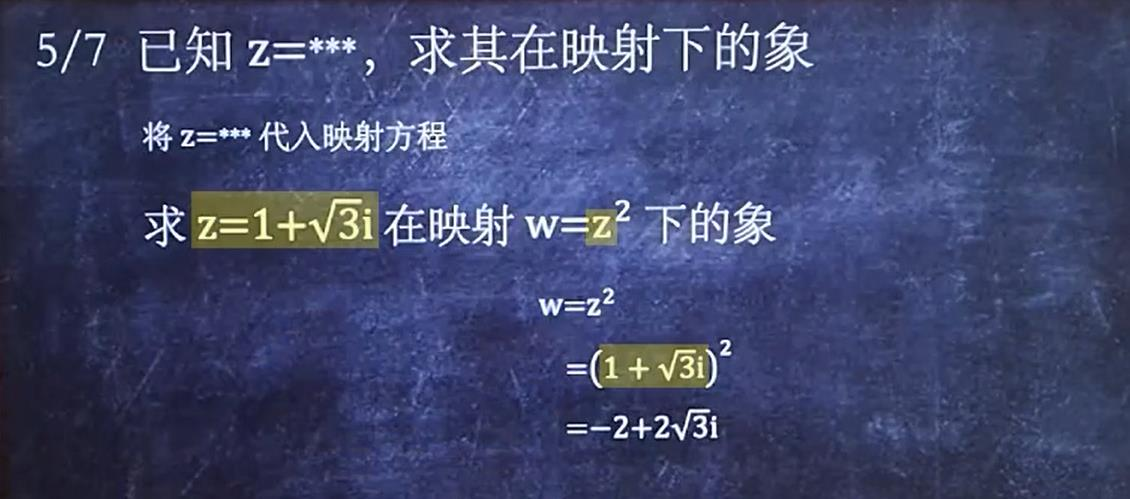相当于对复数的运算。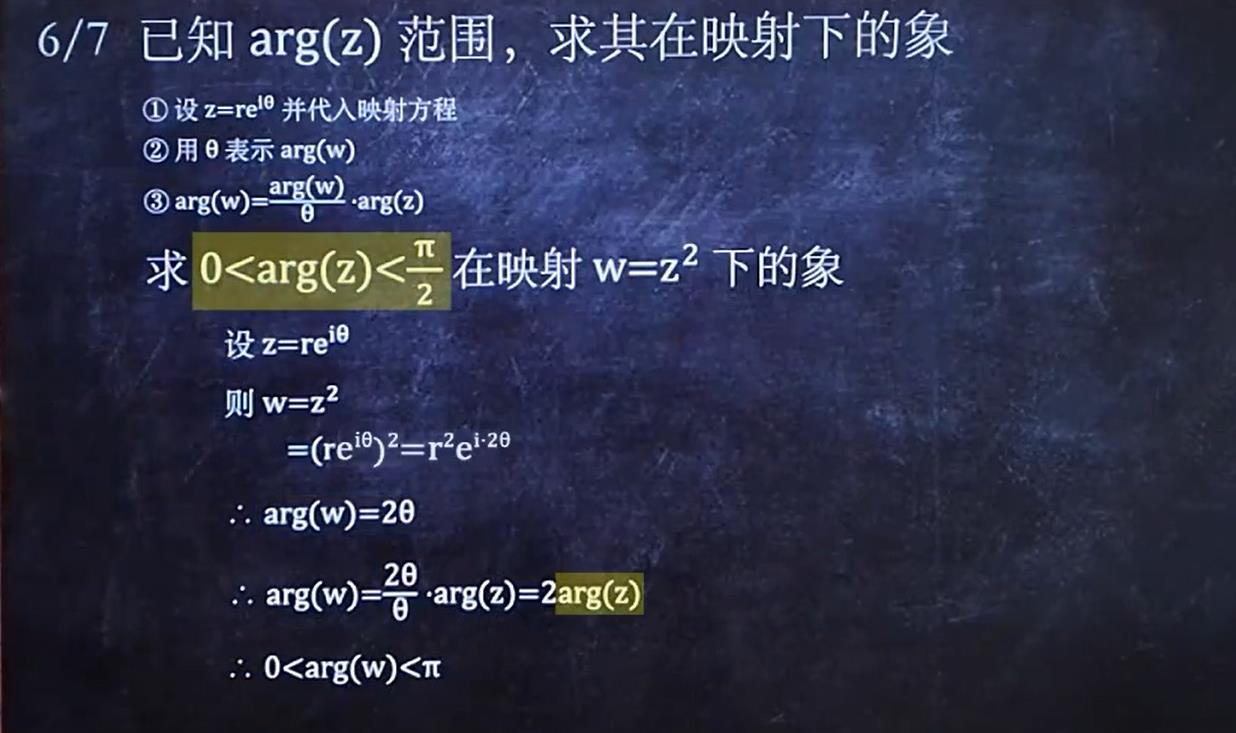相当于求映射后的复数的辐角范围，采用其对数形式比较简单，因为对数形式那直接看出辐角的范围变化。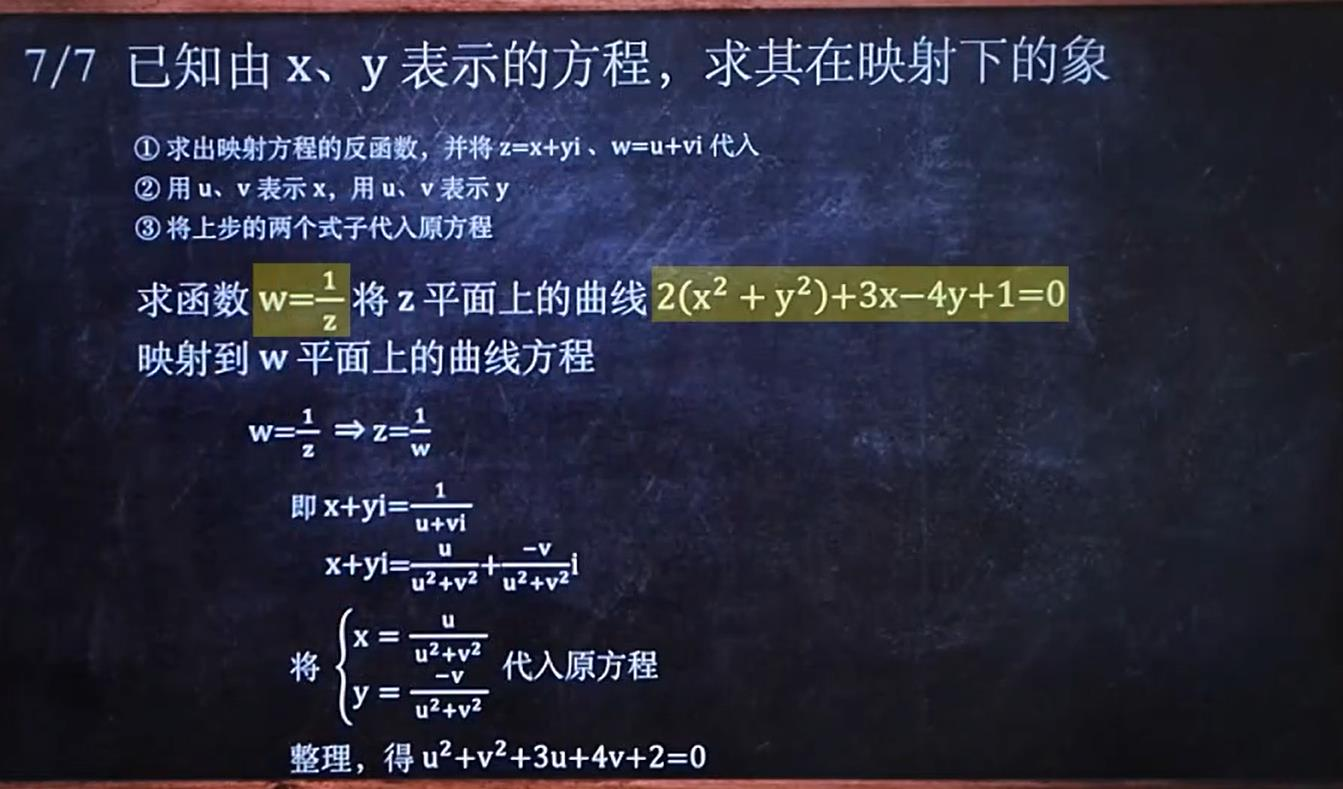此处映射相当于是连接俩个平面，利用函数关系式，z中的x、y对应着w中的u、v，所以主要是用u、v表示出x、y，这也代表着映射的关系转换
PS：复数不能比较大小，除非只有实部

# 三.常见的四种函数

## 1.求复数的三角函数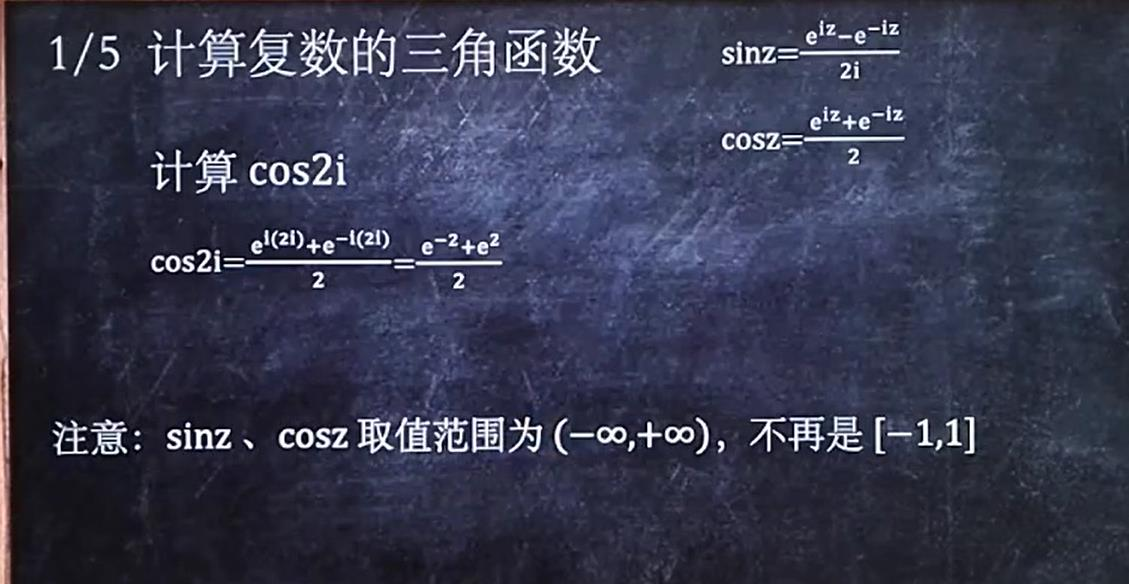求复数的三角函数，把复数看作一个整体，然后套用公式：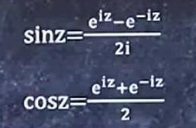即可，注意，此时的三角函数的大小不受限制

## 2.求复数的对数函数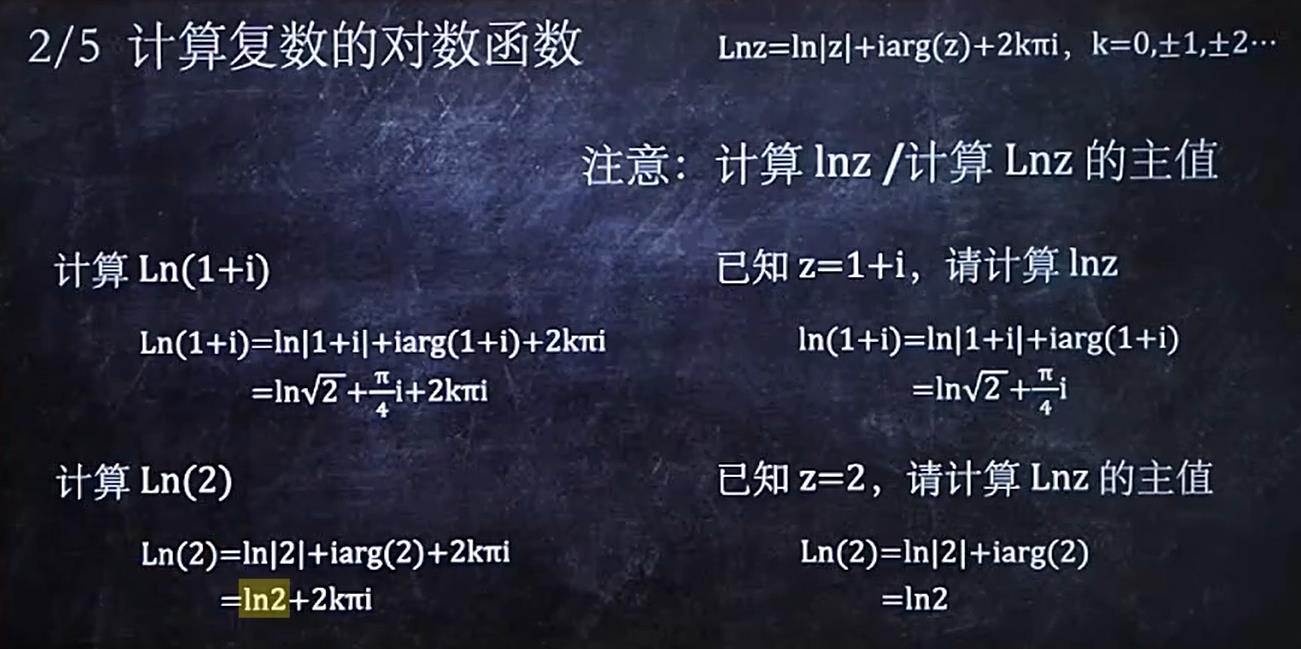在复数的对数中即使要求的复数只有实部，也要按照复数来处理。注意复变函数中的对数书写方式为Ln，其运算也与ln不相同：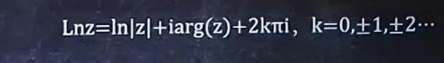复数的对数函数的结果是个周期结果
值得注意的是Ln的主值与ln相同，都是去掉周期性的Ln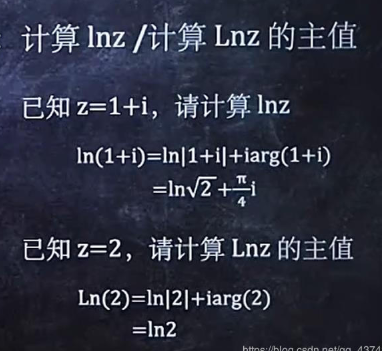## 3.求复数的指数函数

主要围绕此公式展开计算：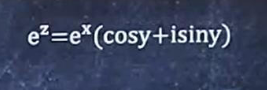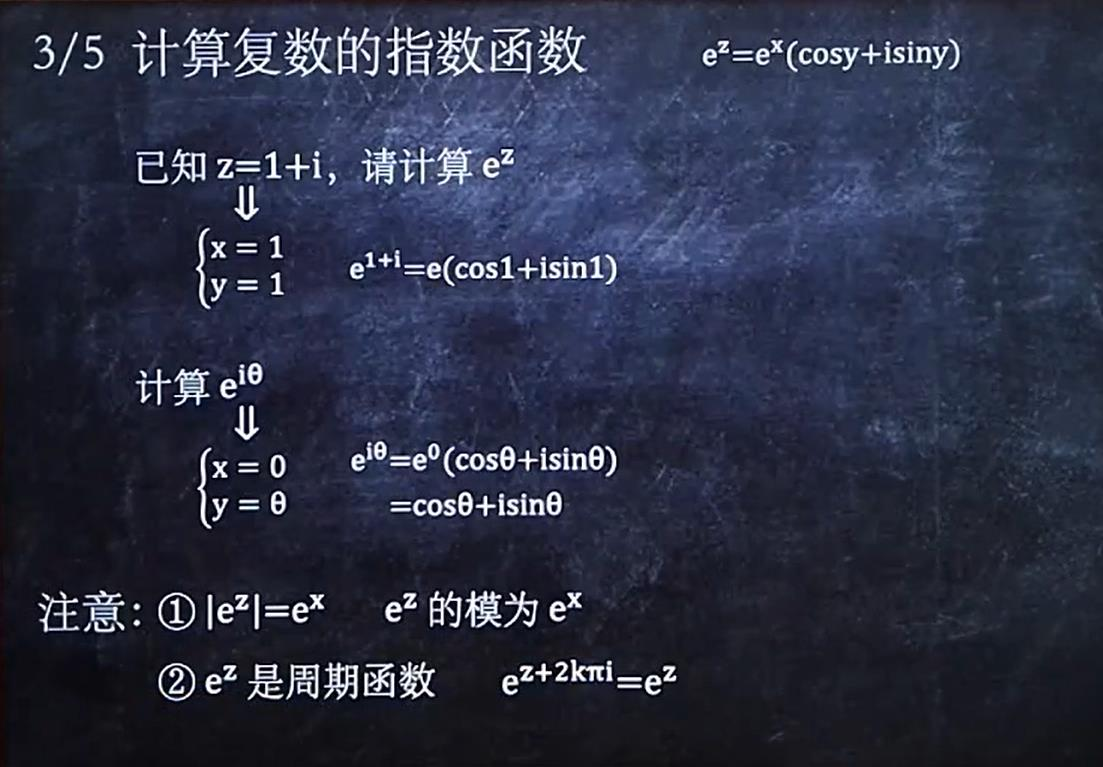不要将指数函数和前面的复数的指数形式混淆，下面练习一道题：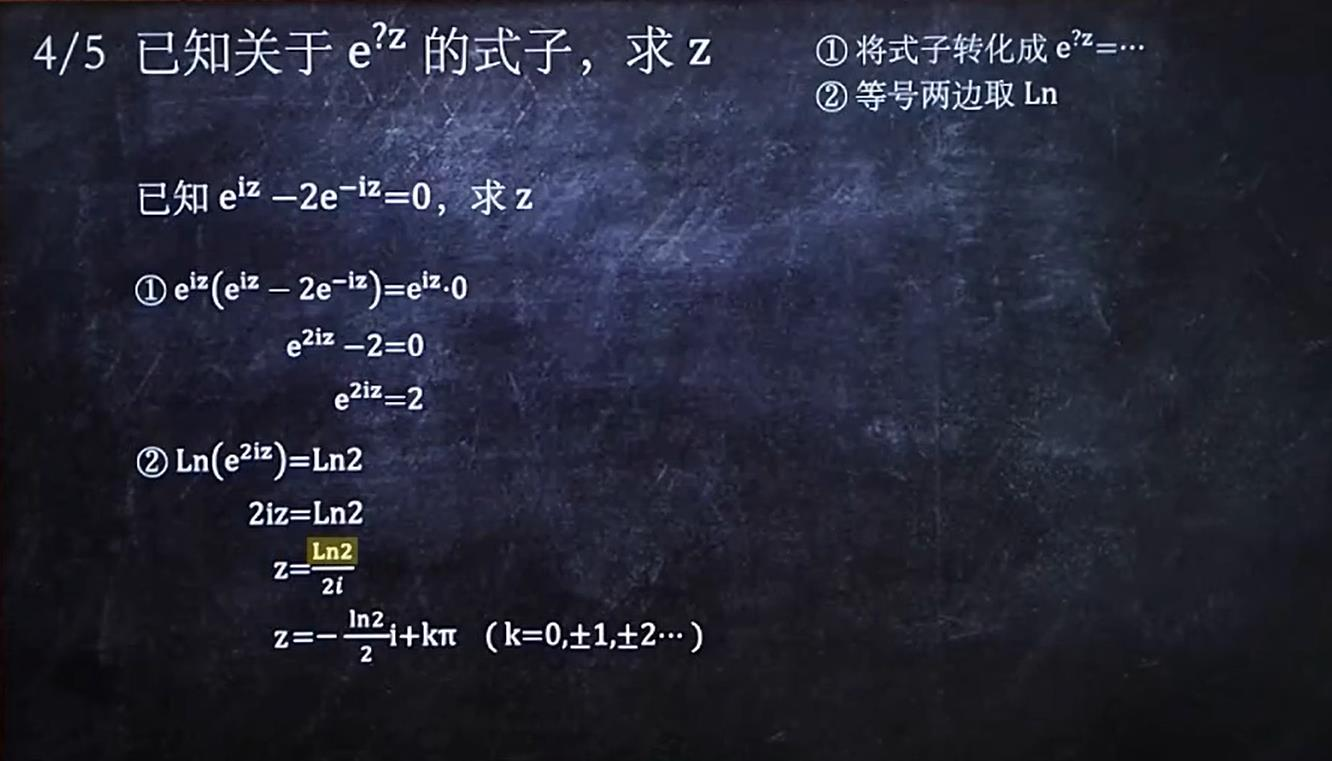此类型题的解法主要是取对数，然后利用对数的运算解题（注意对数函数的周期性）

## 4.求复数的幂函数

幂函数中分为三种情况：
指数为分数的情况与前面的复数开方相对应，而且与开方一样，也有多个结果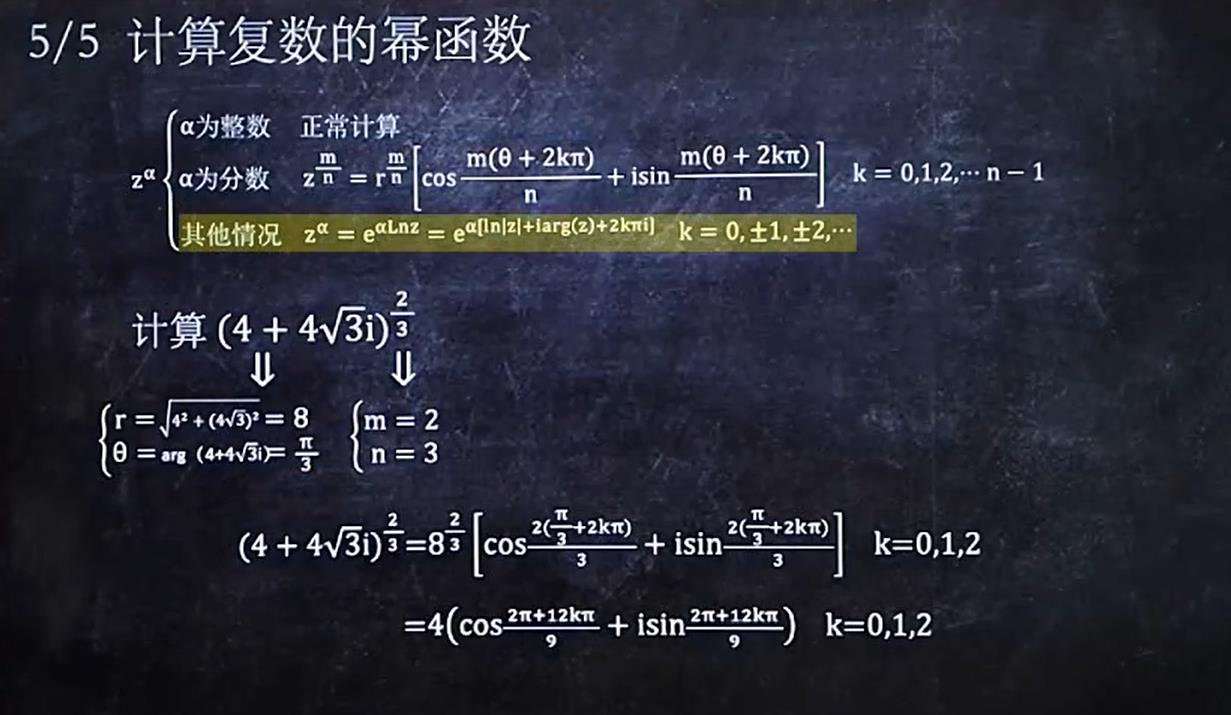当指数为复数时通过取对数Ln的方式来化解，必要时还要利用复数的指数计算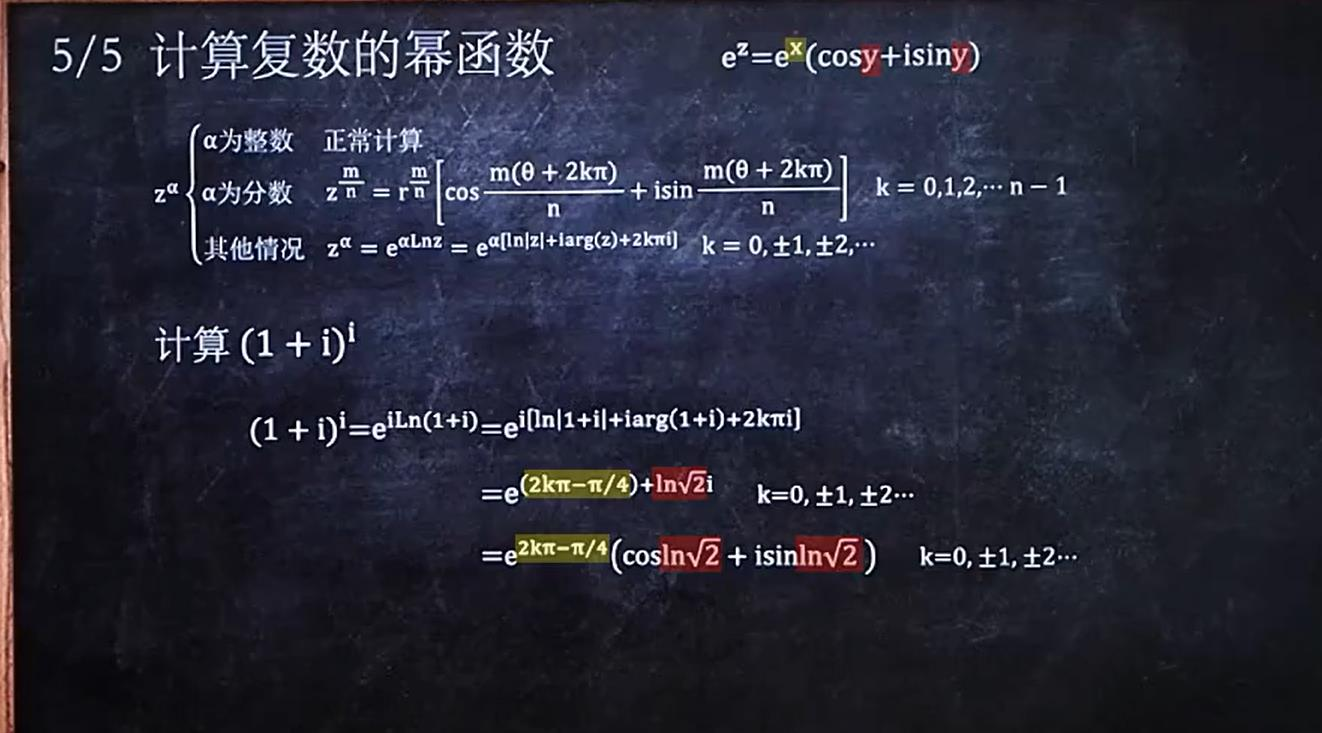注意当指数为负数时，结果与指数是正数时互为倒数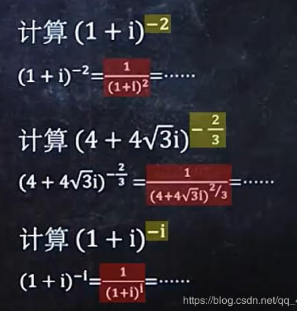# 四.解析调和、求导

## 1.求导数

对复数函数求导主要有俩种类型：
第一种：在u、v中分别对x求导，注意 ：u、v都对x求导，然后相加即可。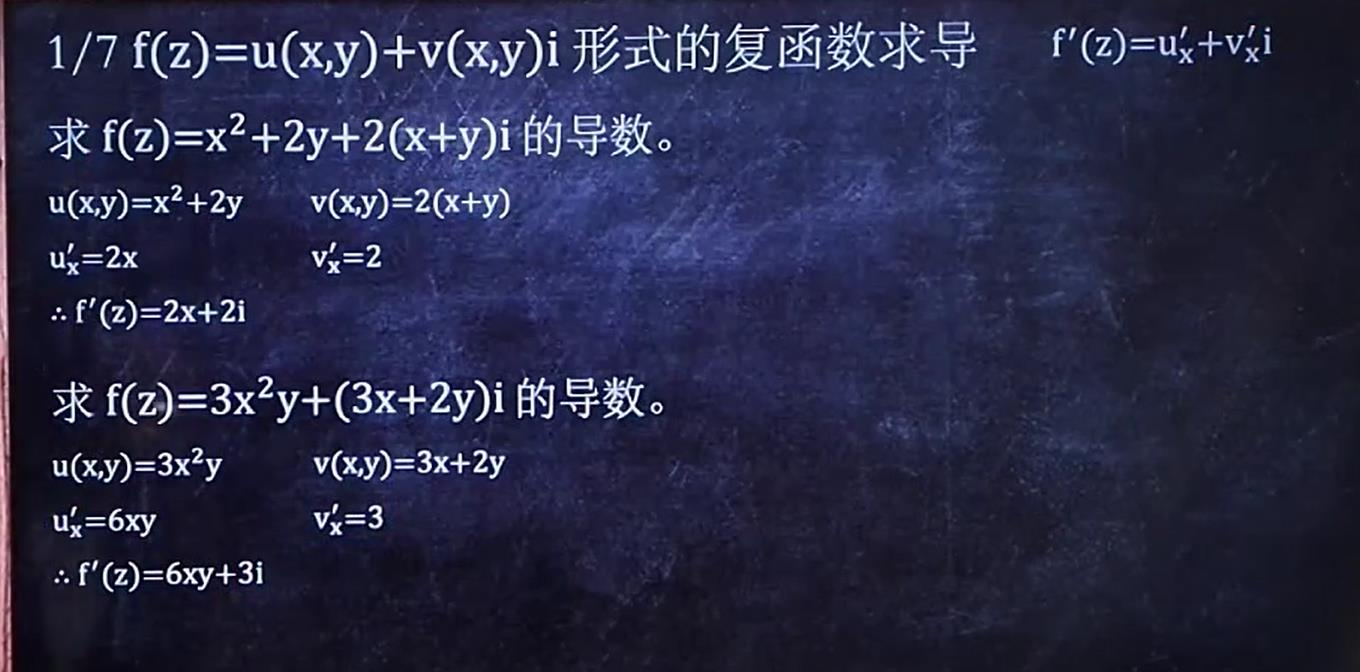第二种：复数以整体的形式出现时就把复数当作实数来处理，而且此时复数的导数运算规则与实数相同，如示：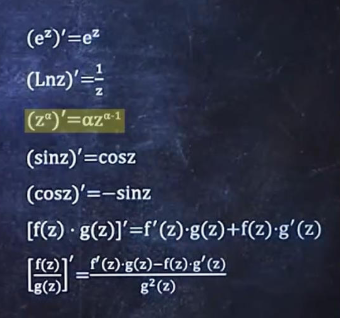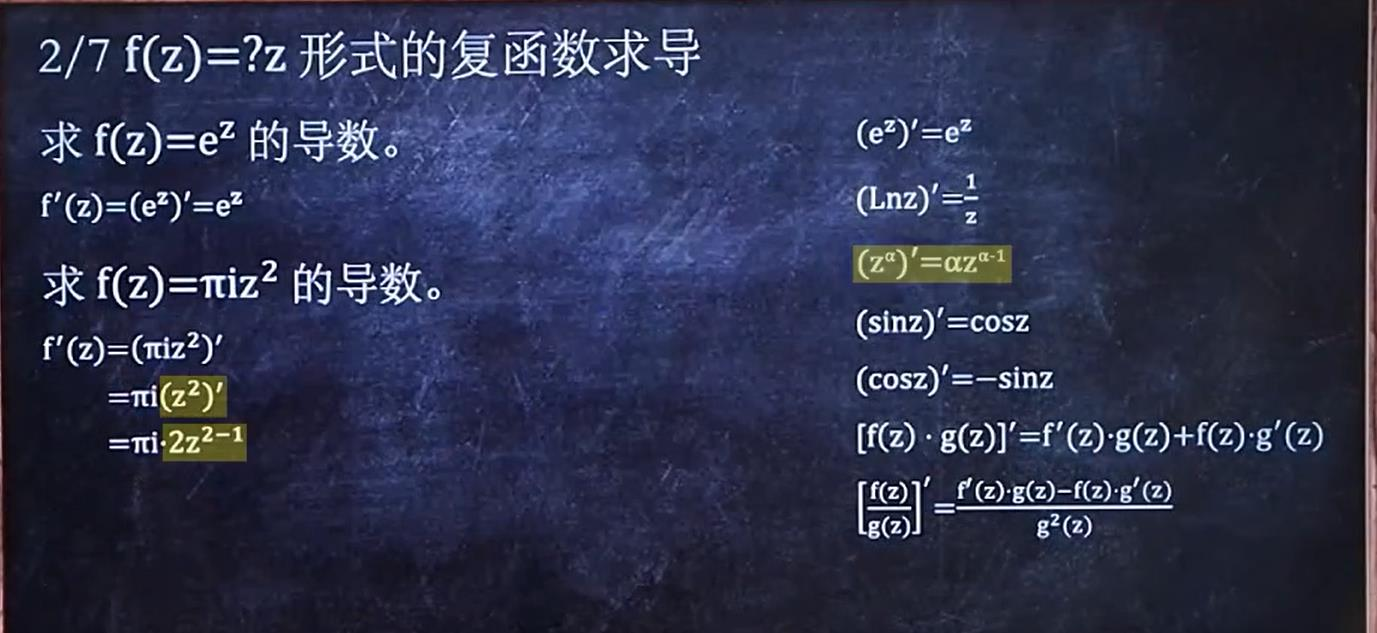## 2.解析、可导、有极限关系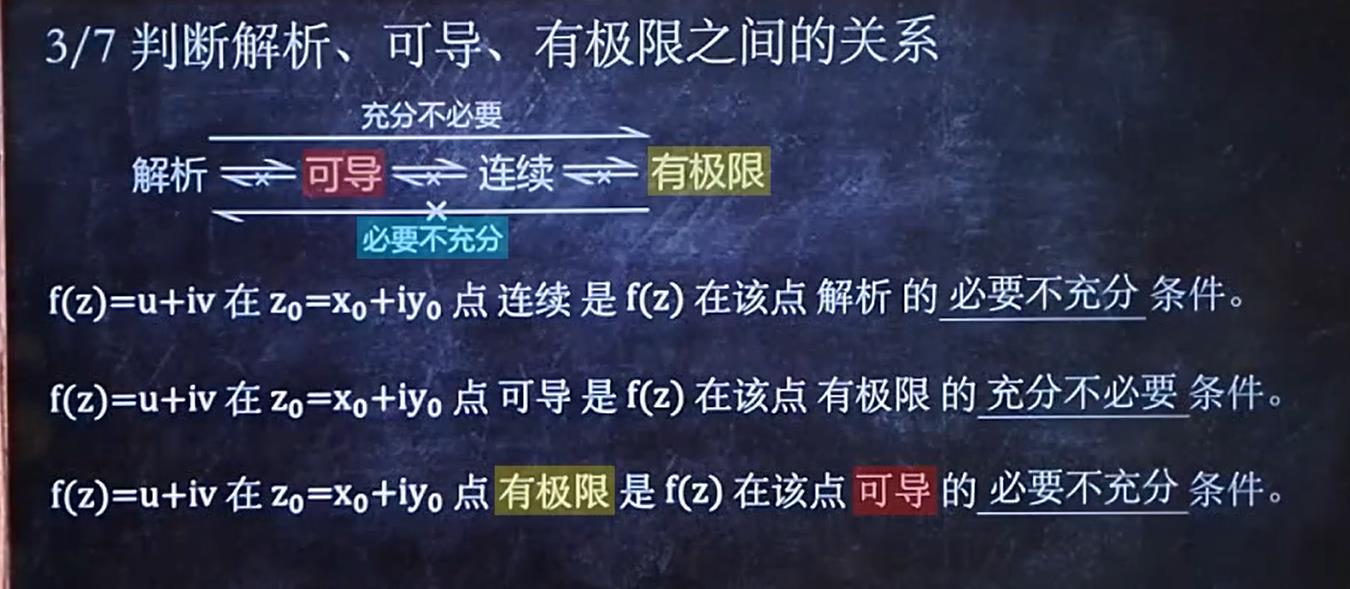## 3.判断可导与解析

判断可导与解析主要看如下公式：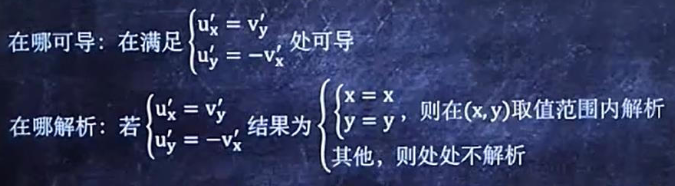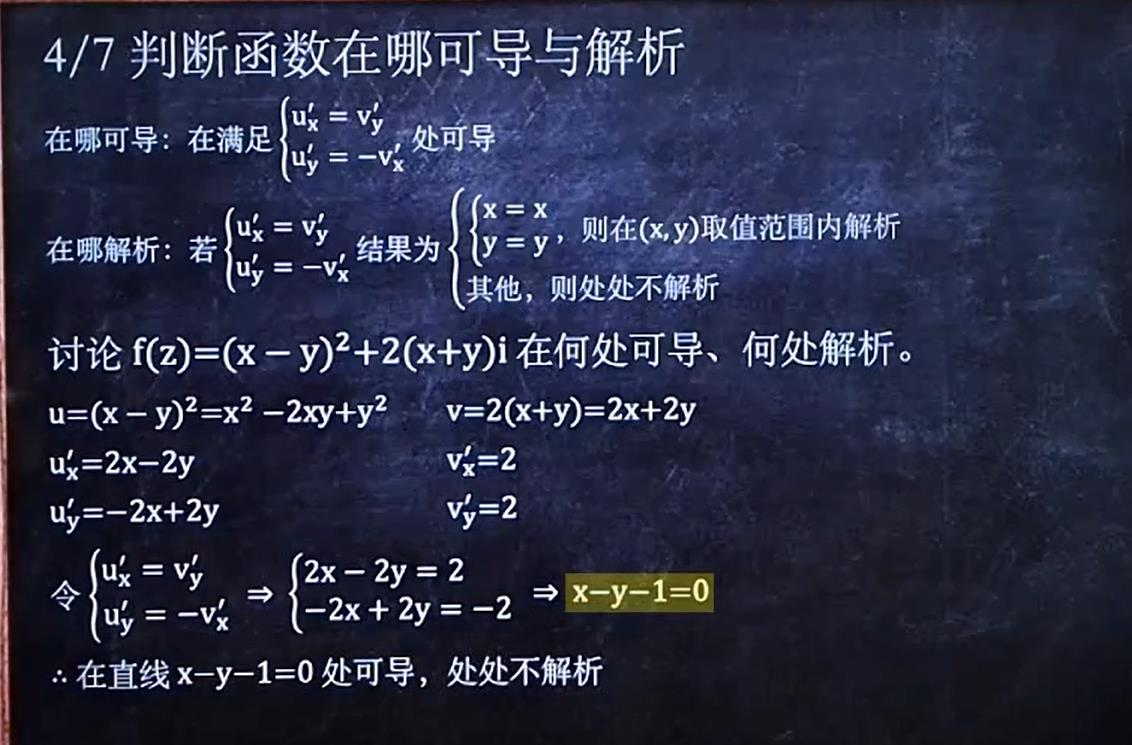练习一道习题：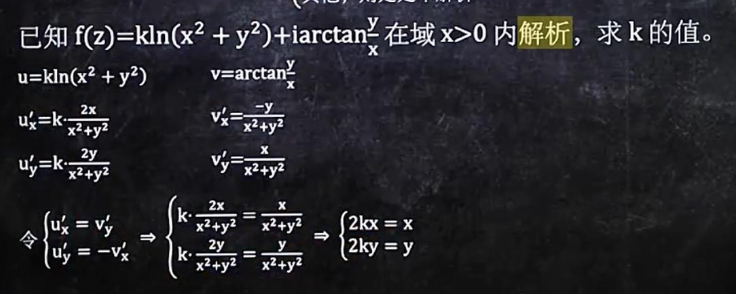## 4.证明调和函数

证明调和函数就俩个条件：当俩个条件都满足时，此函数为调和函数。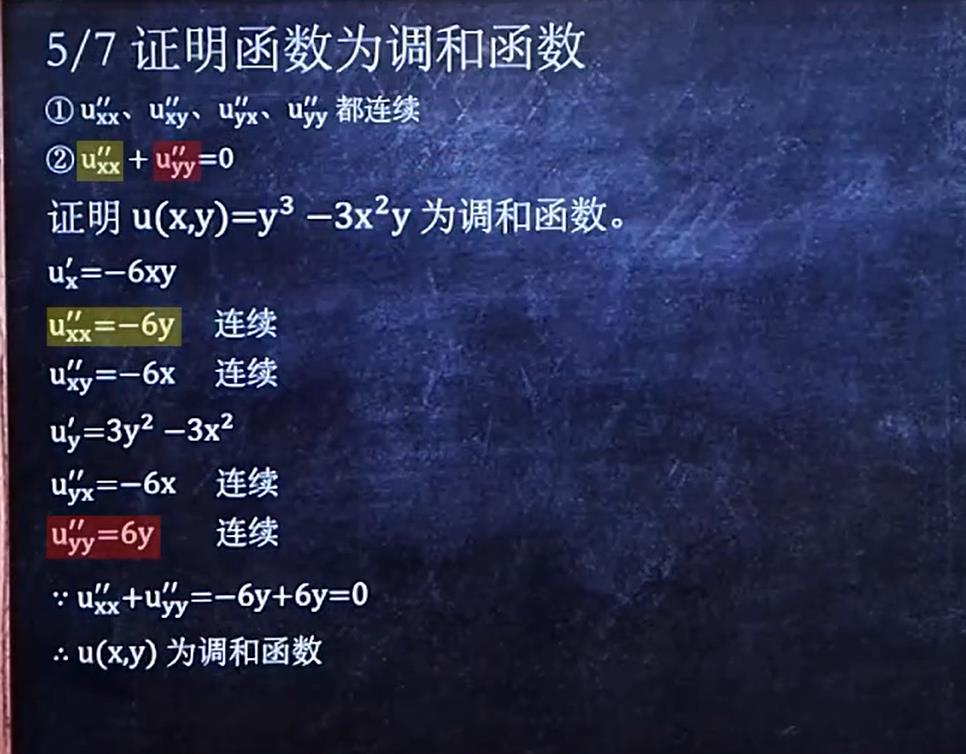## 5.求共轭调和函数

共轭调和函数是建立在调和函数的基础上的，俩个函数分别是对应解析函数的实部和虚部。求共轭调和函数公式如下：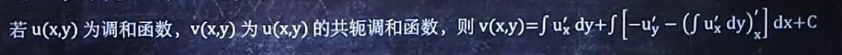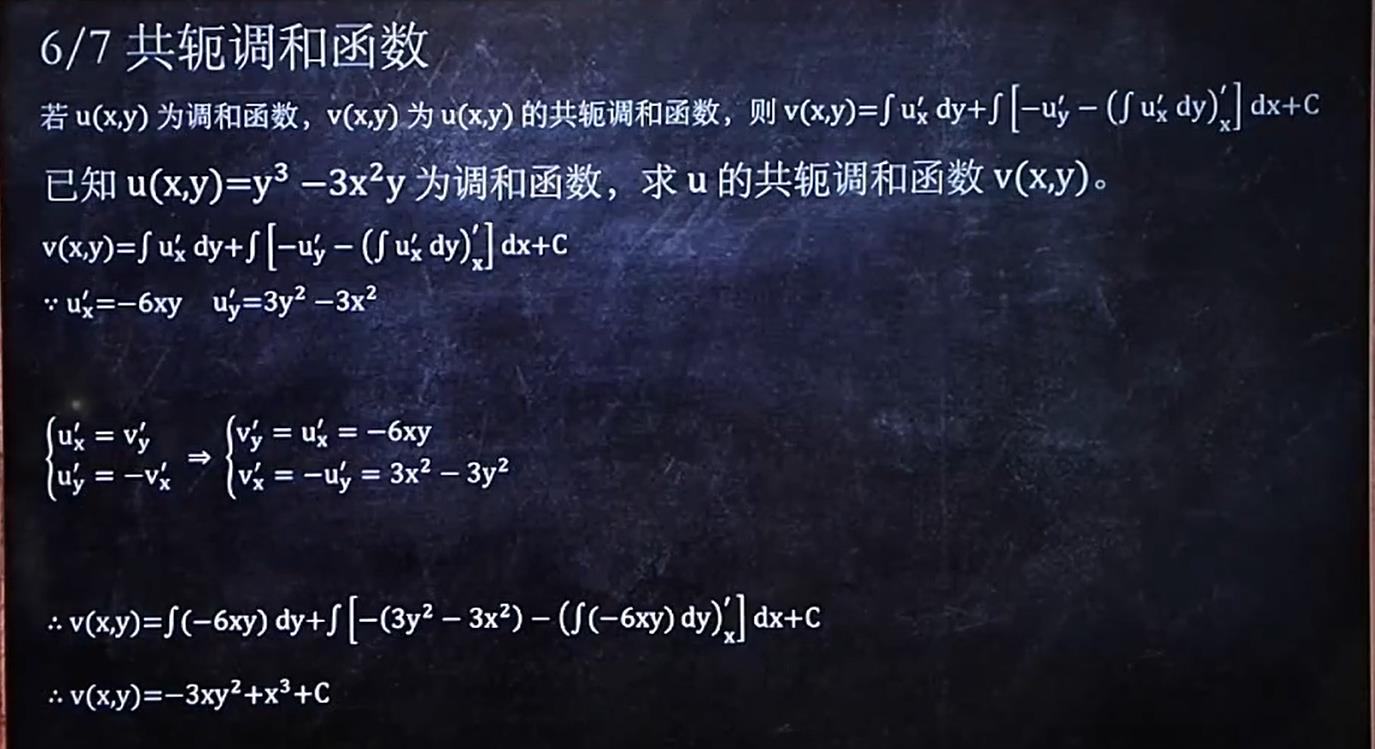## 6.求解析函数

上面说了，调和函数和共轭调和函数相当于解析函数的实部和虚部，所以求解析函数就是求，调和函数和共轭调和函数。
具体求解析函数方法如下：
以（z，0）坐标带入调和函数和共轭调和函数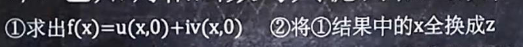# 五.积分

## 1.奇点问题

关于奇点问题无非就是有没有奇点？有几个奇点？已知奇点求积分？

第一种：判断奇点，并求出奇点
就是找出z不能取的值，然后判断不能取的值在不在C的范围内即可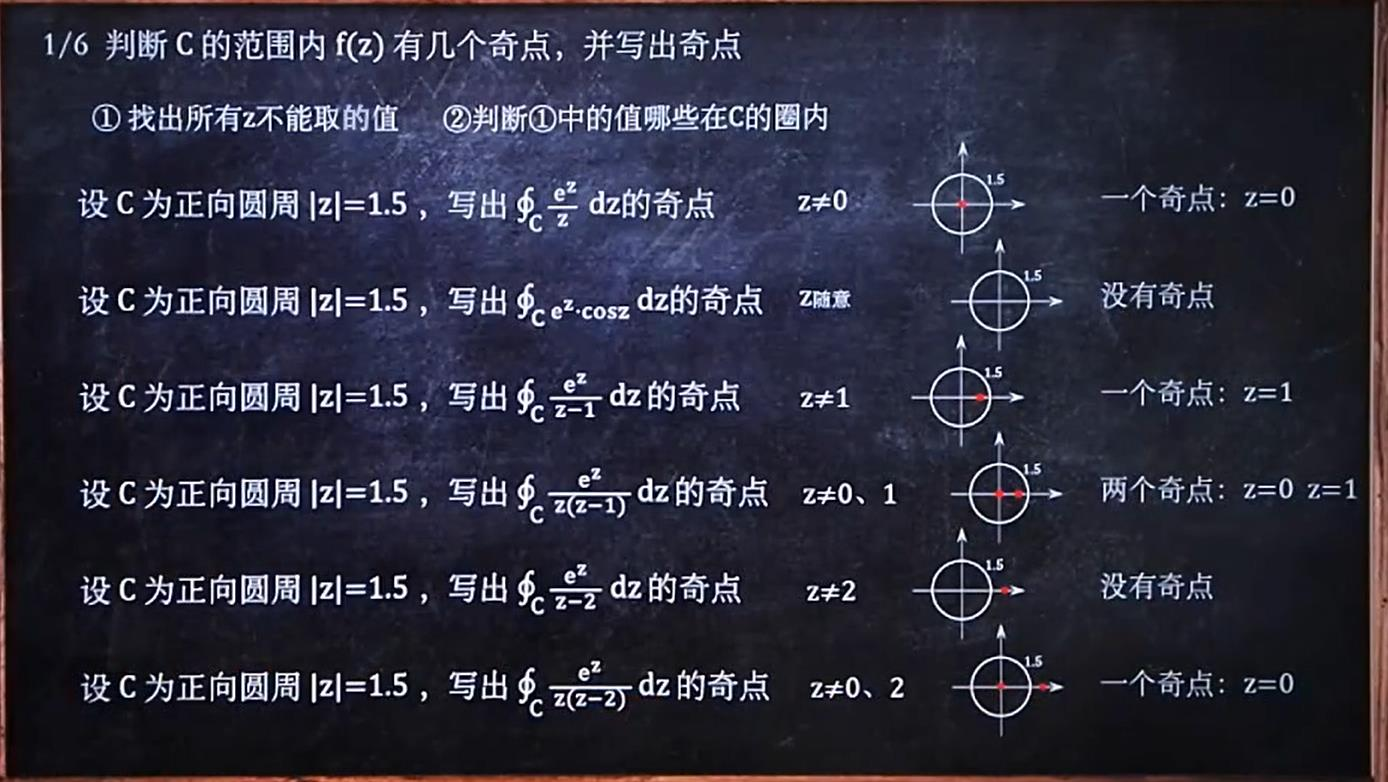第二种：没有奇点时，求积分，都为0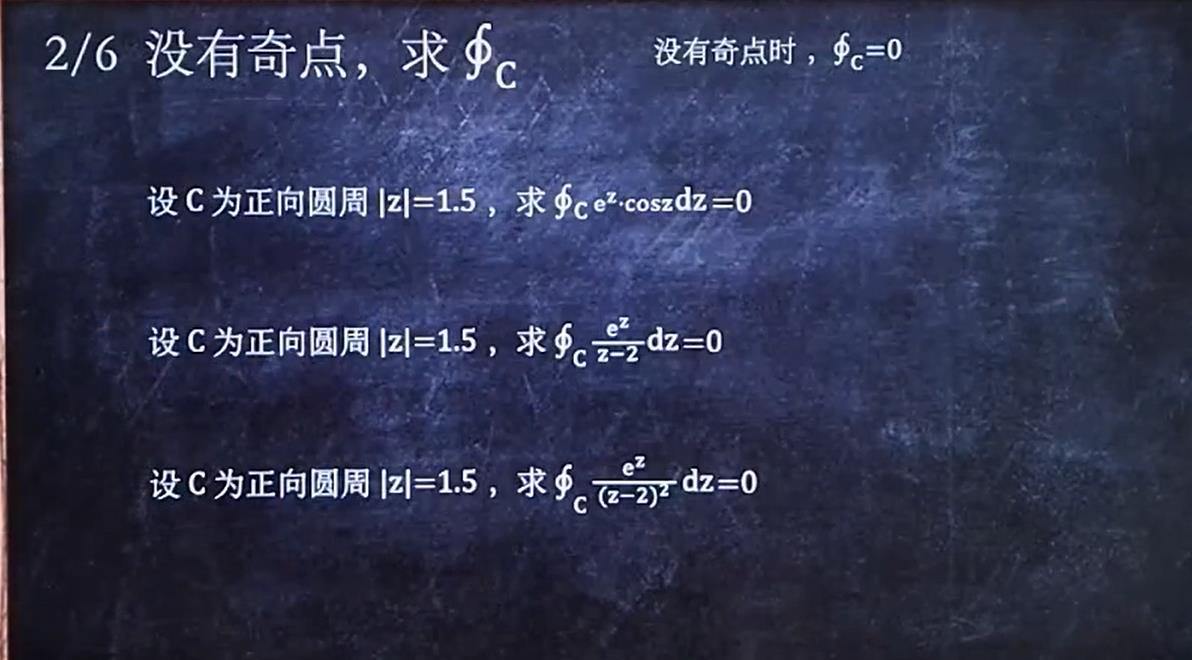第三种：只有一个奇点，求积分，依靠这俩个公式就可以了：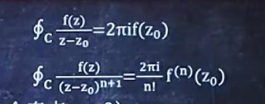Z0就是对应奇点的值，到时候带入就行。要注意分母形态的切换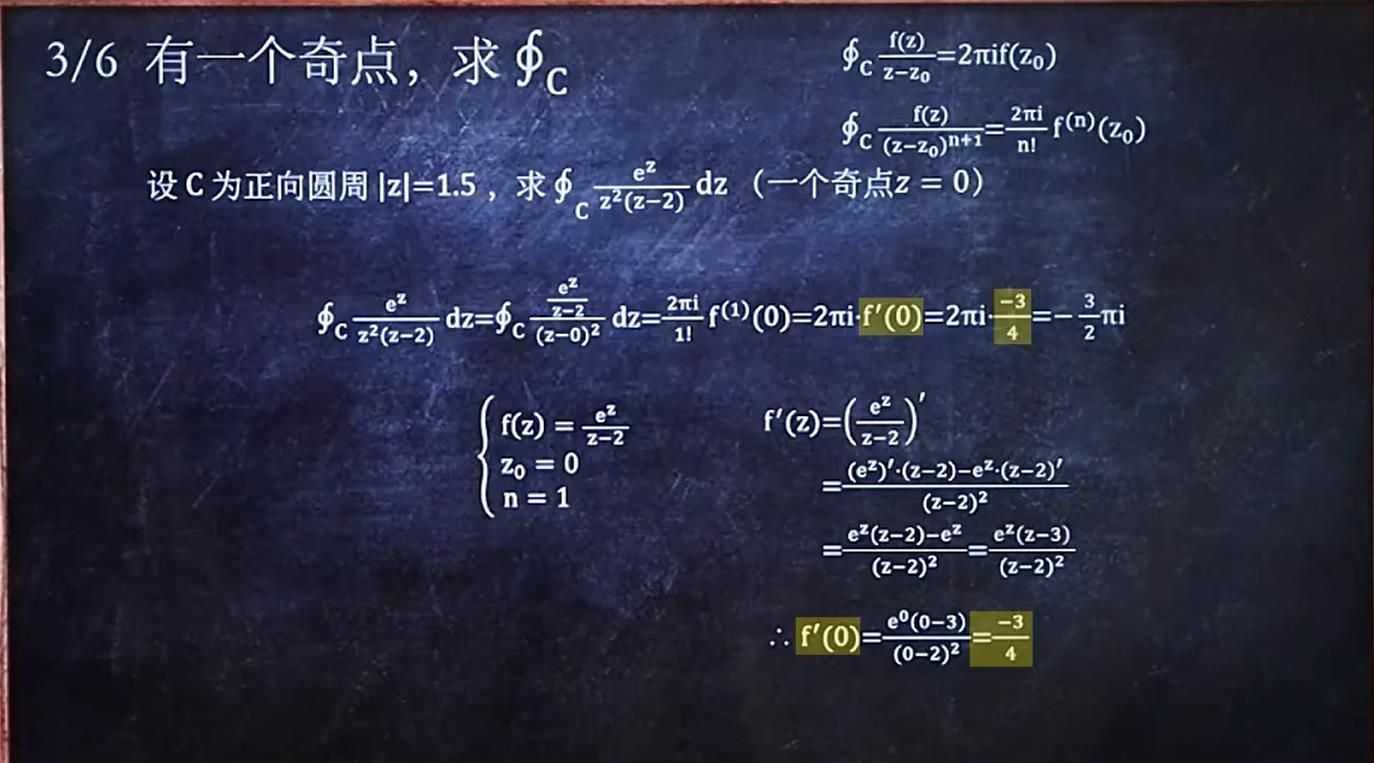第四种：有多个奇点情况下求积分时，将每个奇点的对应的积分求出来之后相加就行。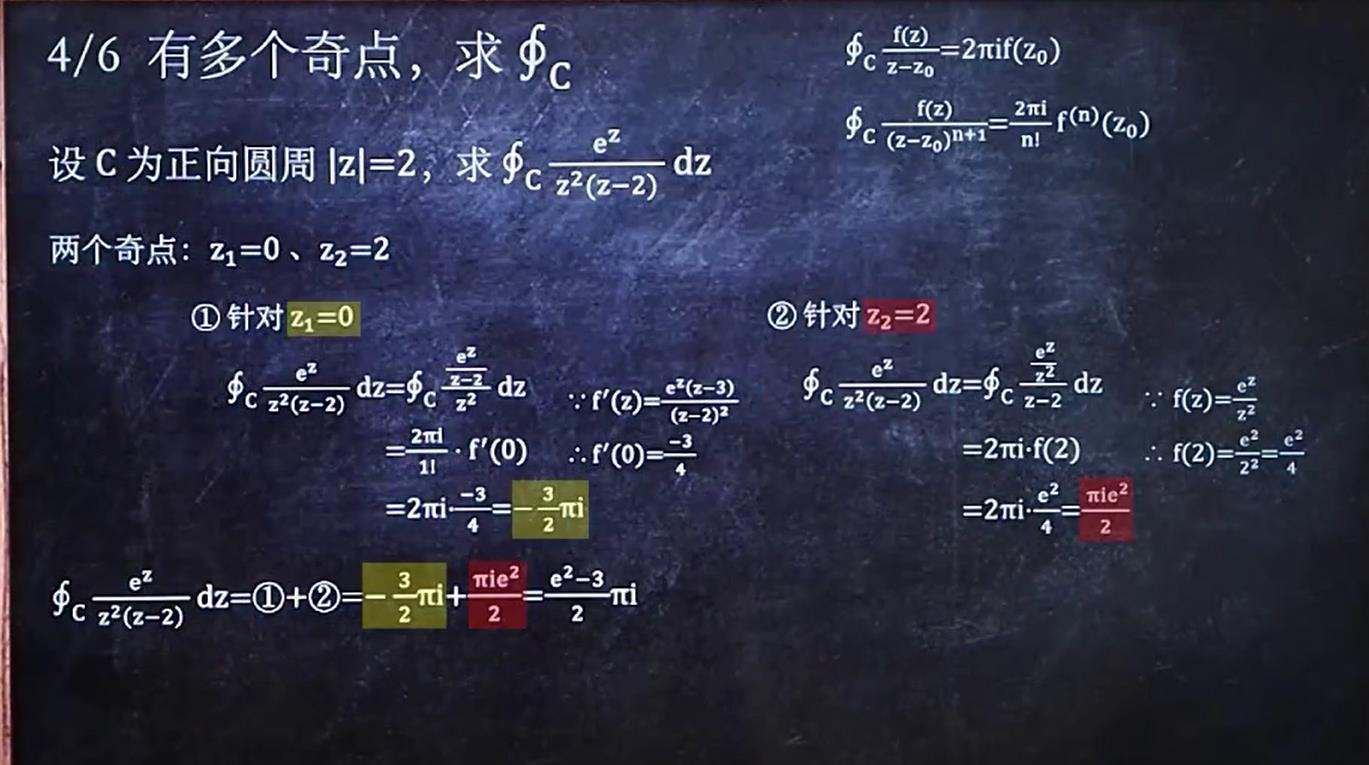## 2.C为线段求积分

相当于用x把z完全替换，需要注意的是C积分路径是有方向的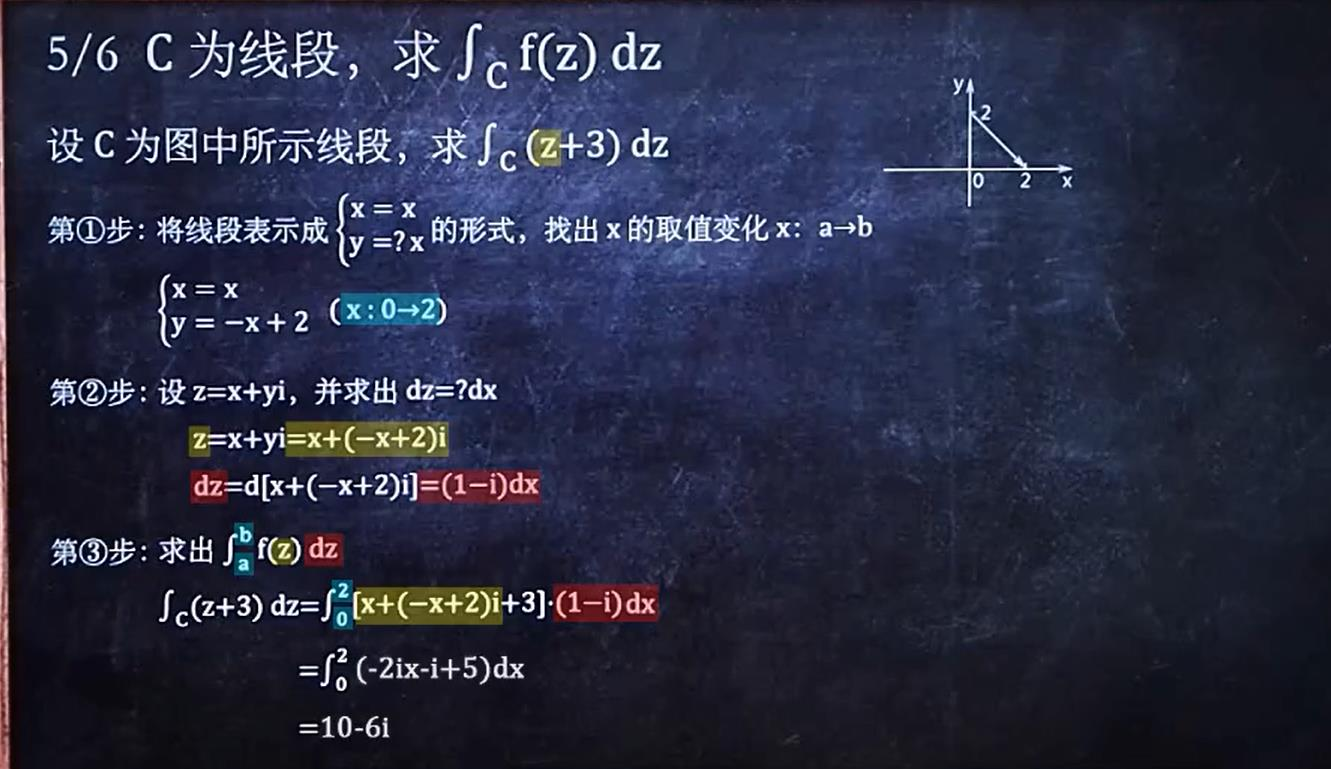C也可以分段来求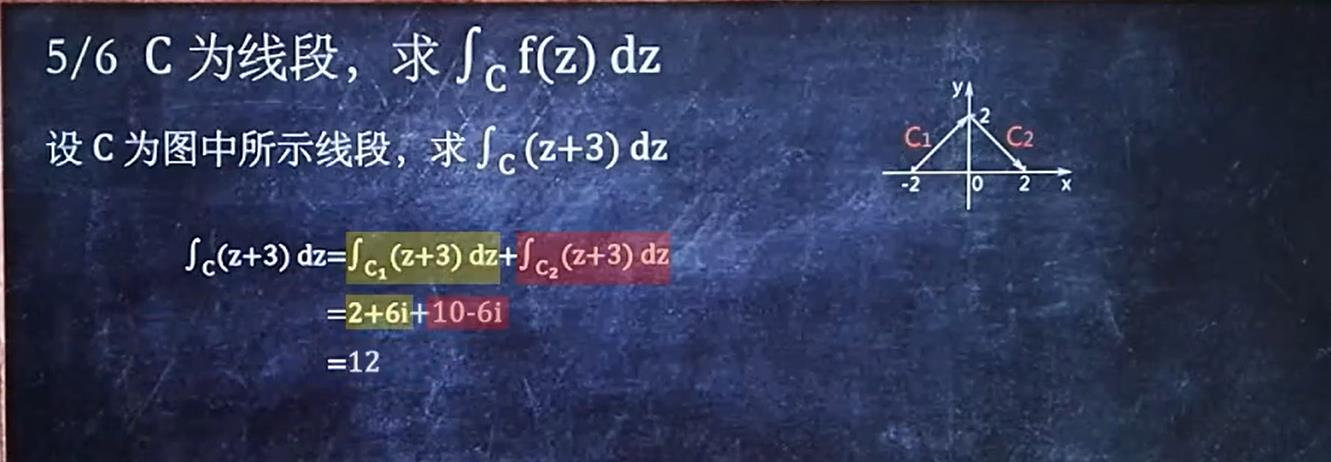## 3.C为弧求积分

C为线段时是用x替换z，C为弧段时要用弧度代替z，实际上也就是个消元思想吧，将代表弧段的参数方程求出来，发现里面唯一的变量就是弧度，所以理所应当的就利用弧度替换x、y、z来简化积分。
需要注意的是：这种情况下经常把复数换为指数形式比较简单，因为涉及到了弧度问题。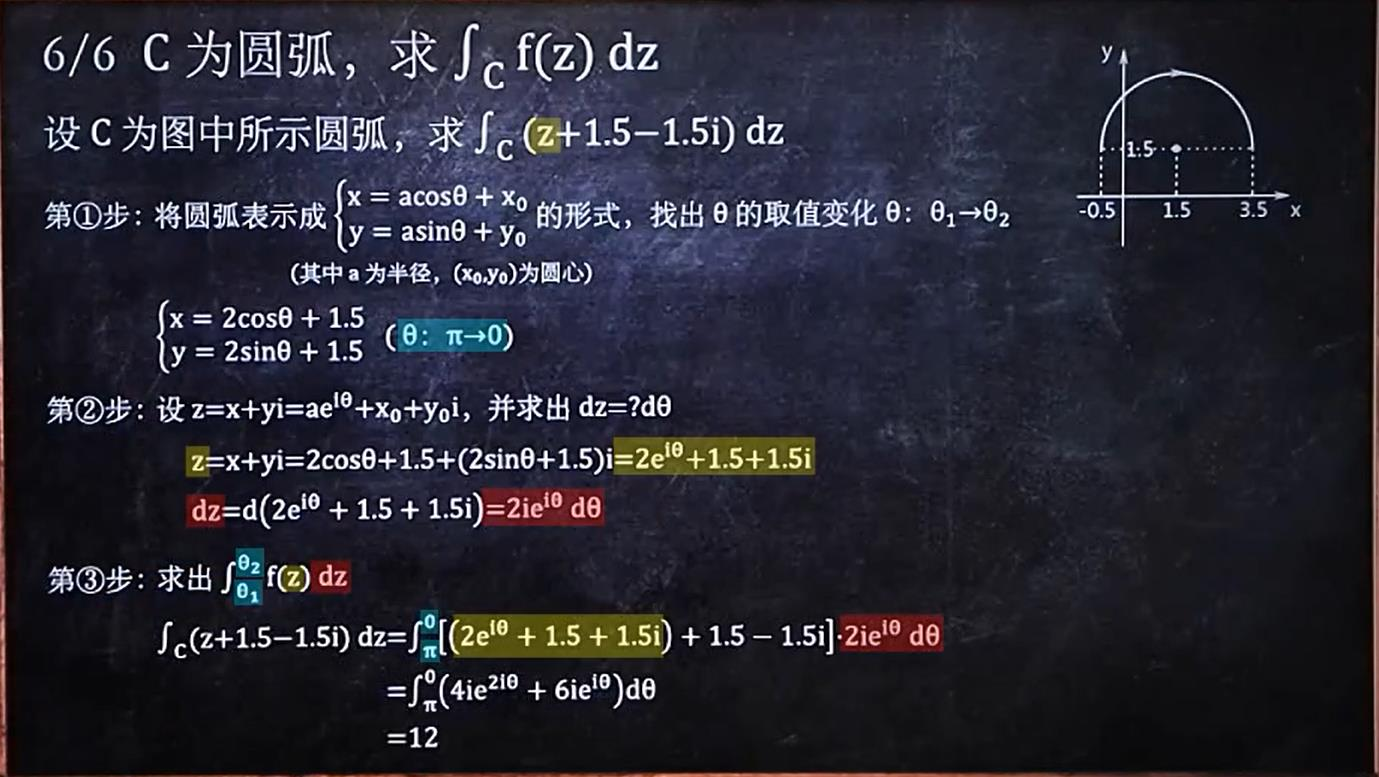展开全文• 复变函数 复变函数定义 对应实变函数，同样也可以建立从复变量映射到复变量的函数。 即可以定义映射 f:S↦S∗f:S\mapsto S^*f:S↦S∗，S,S∗⊆CS,S^*\subseteq\mathbb{C}S,S∗⊆C，对于任一复数 z∈Sz\in Sz∈S，都...

对应实变函数，同样也可以建立从复变量映射到复变量的函数。
即可以定义映射 f : S ↦ S ∗ f:S\mapsto S^* S , S ∗ ⊆ C S,S^*\subseteq\mathbb{C} ，对于任一复数 z ∈ S z\in S ，都对应一个或多个 w ∈ S ∗ w\in S^* . 用记号表示为 w = f ( z ) w=f(z) .
上述表述中， S S 定义集合 S ∗ S^* 函数值集合 S S f f 定义域 w w z z 映象）， z z w w 原象
为了形象地表示复变函数的映射情况，通常用从一个复平面映射到另一个复平面的方式来体现函数的几何特征。

【例 2 - 1 2\verb|-|1 】用图像表示函数 w = z 2 w=z^2 .具体变换式与曲线对应方程如下：第一组方程描述 x - y x\verb|-|y 平面映射到 u - v u\verb|-|v 平面的变换。
第二、三组方程描述 u - v u\verb|-|v 平面上平行于 x , y x,y 两轴的直线对应在 x - y x\verb|-|y 平面中的原象。
当然也可以观察辐角的变化：同时也可以观察 u - v u\verb|-|v 平面上的每个单位小正方形区域对应在 x - y x\verb|-|y 平面中的原象：不难看出，在两复平面内，原象中的两条相交直线形成的夹角在经过变换后没有发生变化。称满足此条件的函数映射本身为 共形映射保形映射 。共形映射和的具体内容会在之后提及。

同一个函数在不同类型的平面内几何特征也不同。上例中若将 x - y x\verb|-|y 平面与 u - v u\verb|-|v 平面都转化为极坐标，可得到下面的图像：容易看出，该例中极坐标下函数的图像变得尤其简单。转化坐标对研究某些函数很有帮助。

同样地，也可以将复变函数用指数函数形式来表示。

【例 2 - 2 2\verb|-|2 】用图像表示函数 w = e z w=e^z .
以下直接给出图像的两复平面上的等值线与辐角的对应关系图像。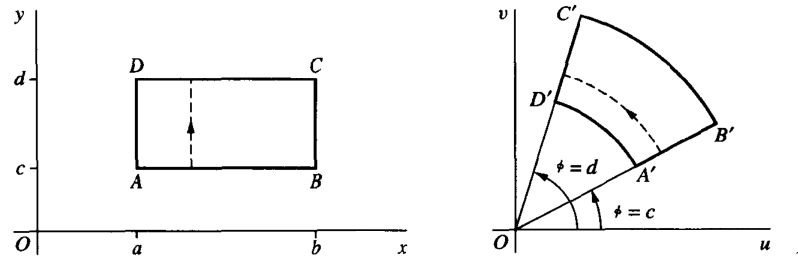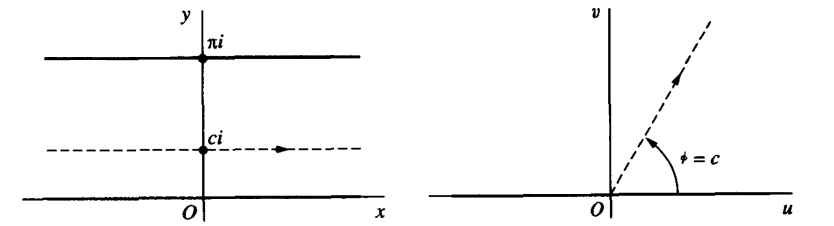复变函数也有反函数的概念。按照实变函数的定义进行类比，可以得到如下定义：
若函数 w = f ( z ) w=f(z) 的定义集合为 z z 平面上的集合 S S ，函数值集合为 w w 平面上的集合 S ∗ S^* 。对于映射 f : S ↦ S ∗ f:S\mapsto S^* S ∗ S^* 上每一点 w w 必对应着 S S 中的一个（或多个）点，由此在 S ∗ S^* 上就确定了一个单值（或多值）函数 z = φ ( w ) z=\varphi(w) ，称为函数 w = f ( z ) w=f(z) 反函数，也称为映射 w = f ( z ) w=f(z) 逆映射

也即：对于任意的 w ∈ S ∗ w\in S^* ，有 w = f [ φ ( w ) ] , w=f[\varphi(w)], 当反函数为单值函数时，也有 z = φ [ f ( z ) ] , z ∈ G . z=\varphi[f(z)],\quad z\in G.

称正映射与逆映射都为单值的函数是 一一的，称对应的集合 S S S ∗ S^* 一一对应 的。

##### Ⅰ .     \bold{Ⅰ}.\ \, 极限的定义

设函数 f f z 0 z_0 的去心邻域中有定义，当 z z 趋近于 z 0 z_0 时， lim ⁡ z → z 0 f ( z ) = w 0 \lim_{z\to z_0}{f(z)}=w_0 w = f ( z ) w=f(z) 可以无限接近于 w 0 w_0 ，只要 z z 足够接近 z 0 z_0 而不等于它。

按照实变函数中极限的 ε - δ \varepsilon\verb|-|\delta 定义，即：对于 ∀   ε > 0 \forall\ \varepsilon>0 ∃   δ > 0 \exist\ \delta>0 s.t. \text{s.t.}     0 < ∣ z − z 0 ∣ < δ \ \ \ 0<|z-z_0|<\delta 时， ∣ f ( z ) − w 0 ∣ < ε . |f(z)-w_0|<\varepsilon.

注意：极限定义中 z z 趋向于 z 0 z_0 的方式是任意的。（类比多元实变函数极限）

##### Ⅱ .     \bold{Ⅱ}.\ \, 极限定理

定理一 【极限唯一定理】 \quad 设函数 f ( z ) f(z) 在一点 z 0 z_0 处存在极限值，那么极限值唯一。

定理二 【极限分部定理】 \quad 设函数 f ( z ) = u ( x , y ) + i v ( x , y ) , A = u 0 + i v 0 , z 0 = x 0 + i y 0 , f(z)=u(x,y)+iv(x,y),\quad A=u_0+iv_0,\quad z_0=x_0+iy_0, 那么 lim ⁡ z → z 0 f ( z ) = A \displaystyle{\lim_{z\to z_0}{f(z)}=A} 的充要条件是 lim ⁡ ( x , y ) → ( x 0 , y 0 ) u ( x , y ) = u 0 , lim ⁡ ( x , y ) → ( x 0 , y 0 ) v ( x , y ) = v 0 ​ . \lim_{(x,y)\to(x_0,y_0)}{u(x,y)}=u_0,\quad\lim_{(x,y)\to(x_0,y_0)}{v(x,y)}=v_0​.
定理三 【极限四则运算】 \quad lim ⁡ z → z 0 f ( z ) = A ,    lim ⁡ z → z 0 g ( z ) = B \displaystyle{\lim_{z\to z_0}{f(z)=A}},\,\,\displaystyle{\lim_{z\to z_0}{g(z)=B}} ，那么
1.   lim ⁡ z → z 0 f ( z ) ± g ( z ) = A ± B    ; \qquad\qquad\qquad\qquad\qquad\ \, 1. \ \displaystyle{\lim_{z\to z_0}{f(z)\pm g(z)=A\pm B}\; ;}

##### Ⅲ .     \bold{Ⅲ}.\ \, 无穷远点的极限

将无穷远点 ∞ \infty 归入复平面，并且使用相关的极限能够带来许多便利。将纳入了无穷远点的复平面称为 扩充复平面

在扩充复平面上表示无穷远点时，可以先想象在复平面原点的正上方存在一个球与之相切于原点。定义球的北极 N N 为球上在原点正上方的点，南极 S S 即为原点 O O ，则原来的复平面上任一点 z z 都能与 N N 相连并交于球面与另一点 P P ，如图所示。由此，我们可以建立 P P z z 的一一对应关系。而北极点 N N 则定义为无穷远点。上述定义的球面被称为 复球面黎曼球面。它是一种对复数平面加上一个无穷远点的扩张。引入无穷远点以后，我们可以在原有极限的定义中，把 z z w w 的适当的领域替换为 ∞ \infty ，并且以下定理成立：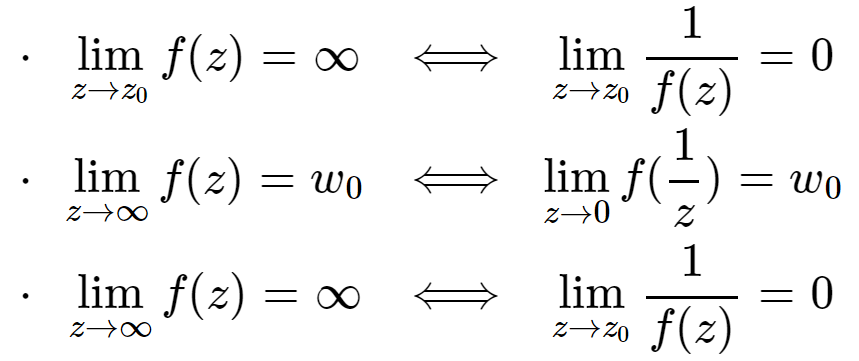上述定理均可用 ε - δ \varepsilon\verb|-|\delta 定义证明。

##### Ⅰ .     \bold{Ⅰ}.\ \, 连续的定义

类比实变函数，复变函数的连续性定义也以极限的概念为基础。

复变函数的连续性定义由以下三个条件共同决定：

• f ( z 0 ) f(z_0) 存在 ; ;
• lim ⁡ z → z 0 f ( z ) \displaystyle{\lim_{z\to z_0}{f(z)}} 存在 ; ;
• lim ⁡ z → z 0 f ( z ) = f ( z 0 ) \displaystyle{\lim_{z\to z_0}{f(z)}=f(z_0)} . .

当然，第三个条件也可以用极限的 ε - δ \varepsilon\verb|-|\delta 定义描述。

如果 f ( z ) f(z) 在区域 D D 范围内处处连续，则称 f ( z ) f(z) D D 内连续。

##### Ⅱ .     \bold{Ⅱ}.\ \, 连续性定理

定理一 【连续分部定理】 \quad 函数 f ( z ) = u ( x , y ) + i v ( x , y ) f(z)=u(x,y)+iv(x,y) z 0 = x 0 + i y 0 z_0=x_0+iy_0 处连续的充要条件是： u ( x , y ) u(x,y) v ( x , y ) v(x,y) ( x 0 , y 0 ) (x_0,y_0) 处连续。

定理二 【连续四则运算】 \quad z 0 z_0 处连续的两个函数 f ( z ) , g ( z ) f(z),g(z) 的和、差、积、商（ z 0 z_0 分母不为 0 0 ） 在 z 0 z_0 处仍连续。

定理三 【连续复合定理】 \quad 若函数 h = g ( z ) h=g(z) z 0 z_0 处连续，函数 w = f ( h ) w=f(h) h 0 = g ( z 0 ) h_0=g(z_0) 处连续，则复合函数 w = f [ g ( z ) ] w=f[g(z)] z 0 z_0 处连续。

定理三 【有界性定理】 \quad 在闭曲线或包括曲线在内的曲线段上连续的函数 f ( z ) f(z) 在曲线上有界。即存在一正数 M M ，在曲线上恒有 ∣ f ( z ) ∣ ≤ M . |f(z)|\le M.

##### Ⅰ .     \bold{Ⅰ}.\ \, 导数的定义

设函数 w = f ( z ) w=f(z) 定义于区域 D D z 0 z_0 D D 中的一点，点 z 0 + Δ z z_0+\Delta z 不出 D D 的范围。如果极限 lim ⁡ Δ z → 0 f ( z 0 + Δ z ) − f ( z 0 ) Δ z \displaystyle{\lim_{\Delta z\to 0}{\frac{f(z_0+\Delta z)-f(z_0)}{\Delta z}}} 存在，那么就说 f ( z ) f(z) z 0 z_0 可导 ，这个极限值称为 f ( z ) f(z) z 0 z_0 导数，记作： f ′ ( z 0 ) = d w d z ∣ z = z 0 = lim ⁡ Δ z → 0 f ( z 0 + Δ z ) − f ( z 0 ) Δ z . f'(z_0)=\frac{dw}{dz}|_{z=z_0}=\lim_{\Delta z\to 0}{\frac{f(z_0+\Delta z)-f(z_0)}{\Delta z}}.

注意：导数定义中 z 0 + Δ z → z 0 z_0+\Delta z\to z_0 （即 Δ z → 0 \Delta z\to 0 ）的方式是任意的，所以复变函数对于导数的限制比对一元实变函数的类似限制要严格很多，并且是复变可导函数有了独特的性质和应用。

f ( z ) f(z) 在区域 D D 内处处可导，则称 f ( z ) f(z) D D 内可导

##### Ⅱ .     \bold{Ⅱ}.\ \, 导数的性质
1. 四则运算
• ( c ) ′ = 0 (c)'=0
• ( z n ) ′ = n z n − 1 (z^n)'=nz^{n-1}
• [ f ( z ) ± g ( z ) ] ′ = f ′ ( z ) ± g ′ ( z ) [f(z)\pm g(z)]'=f'(z)\pm g'(z)
• [ f ( z ) g ( z ) ] ′ = f ′ ( z ) g ( z ) + f ( z ) g ′ ( z ) [f(z)g(z)]'=f'(z)g(z)+f(z)g'(z)
• [ f ( z ) g ( z ) ] ′ = 1 g 2 ( z ) [ g ( z ) f ′ ( z ) − f ( z ) g ′ ( z ) ] \displaystyle{[\frac{f(z)}{g(z)}]'=\frac{1}{g^2(z)}[g(z)f'(z)-f(z)g'(z)]}
• f [ g ( z ) ] ′ = f ′ [ g ( z ) ] g ′ ( z ) {f[g(z)]}'=f'[g(z)]g'(z)
• f ′ ( z ) = 1 φ ′ ( w ) ( w = f ( z ) \displaystyle{f'(z)=\frac{1}{\varphi '(w)}}\quad(w=f(z) z = φ ( w ) z=\varphi(w) 互为反函数 ) )
1. 性质
可导一定连续，连续不一定可导。

复变函数的微分概念在形式上和实变函数的微分概念完全相同。

设函数 w = f ( z ) w=f(z) z 0 z_0 处可导，则 Δ w = f ( z 0 + Δ z ) − f ( z 0 ) = f ′ ( z 0 ) Δ z + ρ ( Δ z ) Δ z . \Delta w=f(z_0+\Delta z)-f(z_0)=f'(z_0)\Delta z+\rho(\Delta z)\Delta z. 其中 lim ⁡ Δ z → 0 ρ ( Δ z ) = 0. \displaystyle{\lim_{\Delta z\to 0}{\rho(\Delta z)}=0.} 因此， ∣ ρ ( Δ z ) Δ z ∣ |\rho(\Delta z)\Delta z| Δ z \Delta z 的高阶无穷小量，而 f ′ ( z 0 ) Δ z f'(z_0)\Delta z 是函数 w = f ( z ) w=f(z) 的线性部分，称为函数 w = f ( z ) w=f(z) 在点 z 0 z_0 处的微分，记作： d w = f ′ ( z 0 ) d z , dw=f'(z_0)dz, f ′ ( z 0 ) = d w d z ∣ z = z 0 . f'(z_0)=\frac{dw}{dz}|_{z=z_0}.

f ( z ) f(z) 在区域 D D 内处处可微，则称 f ( z ) f(z) D D 内可微

从上述过程中可以知道，函数 w = f ( z ) w=f(z) z 0 z_0 处可导与在 z 0 z_0 处可微是等价的。

复变函数中，解析的概念比可导更重要。

如果函数 f ( z ) f(z) z 0 z_0 及其邻域内处处可导，则称 f ( z ) f(z) z 0 z_0 解析 。而函数 f ( z ) f(z) 在区域 D D 内每一点解析，则称 f ( z ) f(z) D D 内解析，或称 f ( z ) f(z) D D 内的一个 解析函数
如果 f ( z ) f(z) z 0 z_0 处不解析，则称 z 0 z_0 f ( z ) f(z) 奇点

由定义我们就直接可以看出：函数在某点可导，不一定在该点解析（邻域不可导）；函数在某点解析，则一定在该点可导。

而根据求导法则，我们也可以得出：

• 在区域 D D 内解析的两个函数 f ( z ) , g ( z ) f(z),g(z) 的和、差、积、商（去除分母为 0 0 的点） 在 D D 内仍解析。
• 若函数 h = g ( z ) h=g(z) D D 内解析，函数 w = f ( h ) w=f(h) h ) h) 平面上的区域 G G 解析。如果对 D D 内的每一个点 z z ，函数 g ( z ) g(z) 的对应值 h h 都属于 G G ，则复合函数 w = f [ g ( z ) ] w=f[g(z)] D D 内解析。

##### Ⅰ .     \bold{Ⅰ}.\ \, 解析的充要条件

与实变函数的全微分类似，复变函数对于一个函数是否解析只用定义判断是不够的。从导数的定义出发，我们可以得到更好的函数解析的充要条件——柯西-黎曼方程。

函数在某点处可导的充要条件 \quad 设函数 f ( z ) = u ( x , y ) + i v ( x , y ) f(z)=u(x,y)+iv(x,y) 定义在区域 D D 内，则 f ( z ) f(z) D D 内一点 z = x + i y z=x+iy 可导的充要条件为： u ( x , y ) u(x,y) v ( x , y ) v(x,y) 在点 ( x , y ) (x,y) 可微，并且在该点满足 柯西-黎曼方程 ∂ u ∂ x = ∂ v ∂ y , ∂ u ∂ y = − ∂ v ∂ x . \frac{\partial u}{\partial x}=\frac{\partial v}{\partial y},\qquad\frac{\partial u}{\partial y}=-\frac{\partial v}{\partial x}.

函数解析的充要条件 \quad 函数 f ( z ) = u ( x , y ) + i v ( x , y ) f(z)=u(x,y)+iv(x,y) 在其定义域 D D 内解析的充要条件为： u ( x , y ) u(x,y) v ( x , y ) v(x,y) D D 内可微，并且满足柯西-黎曼方程。

同时，我们也可以得到可导函数 f ( z ) = u ( x , y ) + i v ( x , y ) f(z)=u(x,y)+iv(x,y) 在点 z = x + i y z=x+iy 处的导数公式： f ′ ( z ) = ∂ u ∂ x + i ∂ v ∂ x = 1 i ∂ u ∂ y + ∂ v ∂ y . f'(z)=\frac{\partial u}{\partial x}+i\frac{\partial v}{\partial x}=\frac{1}{i}\frac{\partial u}{\partial y}+\frac{\partial v}{\partial y}.

当然，上述的讨论只是在直角坐标下的。极坐标下对于函数解析可以利用上述结论，用链式法则推出。

函数在某点处可导的充要条件（极坐标下） \quad 设函数 f ( z ) = u ( r , θ ) + i v ( r , θ ) f(z)=u(r,\theta)+iv(r,\theta) 定义在区域 D D 内，则 f ( z ) f(z) D D 内一点 z = r e i θ   ( z ≠ 0 ) z=re^{i\theta}\ (z\ne 0) 可导的充要条件为： u ( r , θ ) u(r,\theta) v ( r , θ ) v(r,\theta) 在点 ( r , θ ) (r,\theta) 可微，并且在该点满足 柯西-黎曼方程 r ∂ u ∂ r = ∂ v ∂ θ , ∂ u ∂ θ = − r ∂ v ∂ r . r\frac{\partial u}{\partial r}=\frac{\partial v}{\partial \theta},\qquad \frac{\partial u}{\partial \theta}=-r\frac{\partial v}{\partial r}.

此时 f ′ ( z ) f'(z) 可以写为 f ′ ( z ) = e − i θ ( ∂ u ∂ r + i ∂ v ∂ r ) . f'(z)=e^{-i\theta}(\frac{\partial u}{\partial r}+i\frac{\partial v}{\partial r}).

解析函数有许多好的性质，所以也相当重要。之后要学习的初等函数都是在整个复平面上解析的函数。我们把这样的函数称为 整函数

##### Ⅱ .     \bold{Ⅱ}.\ \, 解析函数的性质

以上的内容能够更快速地对函数是否解析作出判别。而知道了一个函数是解析函数后，也能带来一些好的性质。

1. 如果 f ( z ) f(z) 在区域 D D 是解析的，且在区域 D D 内处处满足 f ′ ( z ) = 0 f'(z)=0 ，则 f ( z ) f(z) 在区域 D D 内为一常数。
2. 如果 f ( z ) = u + i v f(z)=u+iv 为一解析函数，且 f ′ ( z ) ≠ 0 f'(z)\ne 0 ，那么曲线组 u ( x , y ) = c 1 u(x,y)=c_1 v ( x , y ) = c 2 v(x,y)=c_2 必互相正交，其中 c 1 , c 2 c_1,c_2 为常数。

第二条性质在上一部分中有所提及。由此可猜测，解析函数对应的映射属于共形映射。而此性质的确成立。

展开全文• 研究了二元复变双解析函数的一非线性边问题。首先给出了二元复变双解析函数的定义，讨论了二元双解析函数的Cauchy积分定理和Cauchy积分公式；其次给出了二元复变双解析函数的Cauchy-Fredholm型积分和Plemelj公式...
• 如果按照一个确定的法则fff，对于DDD中的每一个点zzz，都一个或多个复数www与之对应，则称复变数www是复变数zzz的函数，简称为复变函数，记为 w=f(z) ,z∈Dw=f(z)\ ,\quad z\in D w=f(z) ,z∈D 如果zzz...

# 复变函数（2）-复变函数及其解析性

东风夜放花千树，更吹落，星如雨

### 2.1 复变函数的定义：

设 D D 是复平面上一个非空点集。如果按照一个确定的法则 f f ，对于 D D 中的每一个点 z z ，都有一个或多个复数 w w 与之对应，则称复变数 w w 是复变数 z z 的函数，简称为复变函数，记为
w = f ( z )   , z ∈ D w=f(z)\ ,\quad z\in D  如果 z z 的一个值对应于 w w 的一个值，那么称函数 f ( z ) f(z) 是单值的，如果 z z 的一个值对应着 w w 的多个值，那么称函数 f ( z ) f(z) 是多值的。
复变函数也可以和两个二元实函数 u ( x , y )   ,   v ( x , y ) u(x,y)\ ,\ v(x,y) 联系起来，设 w = u + v i w=u+vi z = x + y i z=x+yi ，则
w = u + v i = f ( z ) = f ( x + y i ) = u ( x , y ) + v ( x , y ) i w=u+vi=f(z)=f(x+yi)=u(x,y)+v(x,y)i  复变函数可以类比实函数定义其函数特征。

### 2.2 指数函数：

对于复变数 z = x + y i z=x+yi ，称复变数 w = e x ( c o s y + i s i n y ) w=e^x(cosy+isiny) 为复变数 z z 的指数函数，记作 w = e z w=e^z ，即
w = e z = e x ( c o s y + i s i n y ) w=e^z=e^x(cosy+isiny) { ∣ e z ∣ = e x A r g ( e z ) = y + 2 k π ( k = 0 , ± 1 , ± 2 , … ) \left\{\begin{aligned} & |e^z|=e^x \\ & Arg(e^z)=y+2k\pi\quad (k=0,\pm1,\pm 2,\ldots)\end{aligned}\right.  指数函数是单值函数，且在复平面上处处有定义。
（注：这里单值函数是指在 z = x + y i z=x+yi 这样的实部虚部表示法下，函数值 w = e z w=e^z 有唯一确定的实部和虚部。对于虚数的指数表示法 A r g ( e z ) = y + 2 k π Arg(e^z)=y+2k\pi ，在任何情况下根据定义辐角的取值都是无穷多的，所以不利用复变数的指数和三角表示法来判断函数的单值和多值性。）
复指数函数具有以下性质：
e 0 = 1 e^0=1 e z ≠ 0 e^z\ne0 e z 1 + z 2 = e 1 z e 2 z e^{z_1+z_2}=e^z_1e^z_2 e − z = 1 e z e^{-z}=\frac{1}{e^z} e z ‾ = e z ‾ \overline{e^z}=e^{\overline{z}} e 2 k π i = 1 e^{2k\pi i}=1

### 2.3 对数函数

指数函数的反函数，即满足方程
e w = z ( z ≠ 0 ) e^w=z\quad (z\ne 0)  的复变数 w w 称为复变数 z z 的对数函数，记作 w = L n   z w=Ln\ z
对于 w = u + v i w=u+vi
∣ z ∣ = ∣ e w ∣ = e u |z|=|e^w|=e^u A r g   z = A r g   e w = v Arg\ z=Arg\ e^w=v  所以
u = l n ∣ z ∣   , v = A r g   z u=ln|z|\ ,\quad v=Arg\ z L n   z = l n ∣ z ∣ + i A r g   z ( z ≠ 0 ) Ln\ z=ln|z|+iArg\ z\quad(z\ne 0) w = L n   z = l n ∣ z ∣ + i a r g   z + 2 k π i ( k = 0 , ± 1 , ± 2 , … ) w=Ln\ z=ln|z|+iarg\ z+2k\pi i\quad (k=0,\pm1,\pm 2,\ldots)  可以看出对数函数可以得出多种实部和虚部表示，所以对数函数是多值函数。给定确定 k = k 0 k=k_0 时，可以得到对数函数的一个分支，对数函数的每一个分支都是单值函数。
k = 0 k=0 时的分支称为对数函数 w = L n   z w=Ln\ z 的主分支，对应的函数值为函数主值，记为 l n   z ln\ z ，有
l n   z = l n ∣ z ∣ + i a r g   z ( z ≠ 0 ) ln\ z=ln|z|+iarg\ z\quad (z\ne 0)
对数函数有如下性质
L n ( z 1 z 2 ) = L n   z 1 + L n   z 2 Ln(z_1z_2)=Ln\ z_1+Ln\ z_2 L n ( z 1 z 2 ) = L n   z 1 − L n   z 2 Ln(\frac{z_1}{z_2})=Ln\ z_1-Ln\ z_2  等式两边都是多值函数，等号意味着两段可能取得的函数值全体相同。同理，下面的式子不成立
L n   z n ≠ n L n   z Ln\ z^n\ne nLn\ z L n   z n ≠ 1 n L n   z Ln\ \sqrt[n]{z}\ne \frac{1}{n}Ln\ z

### 2.4 幂函数

设 a a 是复常数，对于复变数 z ≠ 0 z\ne 0 ，称复变数 w = e a L n   z w=e^{aLn\ z} 为复变数 z z 的幂函数，即
w = z a = e a L n   z w=z^a=e^{aLn\ z}  当 a a 为正实数，且 z = 0 z=0 时，规定 z a = 0 z^a=0
指数函数的取值性需要分情况讨论。
(1)当 a = n a=n 为整数时
w = z n = e n L n   z = e n [ l n ∣ z ∣ + i ( a r g   z + 2 k π ) ] = e n l n ∣ z ∣ + ( n a r g   z ) i + 2 n k π i = e n l n ∣ z ∣ + ( n a r g   z ) i ( k = 0 , ± 1 , ± 2 , … ) w=z^n=e^{nLn\ z}=e^{n[ln|z|+i(arg\ z+2k\pi)]}=e^{nln|z|+(narg\ z)i+2nk\pi i}=e^{nln|z|+(narg\ z)i}\quad (k=0,\pm1,\pm 2,\ldots)  所以此时幂函数为单值函数，当 n > 0 n>0 时在复平面上处处有定义。当 n < 0 n<0 时，在复平面除 z = 0 z=0 点外处处有定义。
(2)当 a = p q a=\frac{p}{q} p p q q 为互质的整数， q > 0 q>0 ）为有理数时
w = z a = e p q L n   z = e p q [ l n ∣ z ∣ + i ( a r g   z + 2 k π ) ] = e p q l n ∣ z ∣ + ( p q a r g   z ) i + 2 p q k π i w=z^a=e^{\frac{p}{q}Ln\ z}=e^{\frac{p}{q}[ln|z|+i(arg\ z+2k\pi)]}=e^{\frac{p}{q}ln|z|+(\frac{p}{q}arg\ z)i+2\frac{p}{q}k\pi i} = e p q l n ∣ z ∣ [ c o s p q ( a r g   z + 2 k π ) + i s i n p q ( a r g   z + 2 k π ) ) ] ( k = 0 , ± 1 , ± 2 , … ) =e^{\frac{p}{q}ln|z|}[cos\frac{p}{q}(arg\ z+2k\pi)+isin\frac{p}{q}(arg\ z+2k\pi))]\quad (k=0,\pm1,\pm 2,\ldots)
根据三角函数的周期性，函数 w = z p q w=z^{\frac{p}{q}} 具有 q q 个不同的值，当 k = 0 , 1 , … , q − 1 k=0,1,\ldots,q-1 时可以取得。
(3)当 a a 为无理数或虚数时，幂函数是无穷多值的，在复平面上除了 z = 0 z=0 外处处有定义，每一个单值分支对应于 L n   z Ln\ z 的一个单值分支。

### 2.5 三角函数

分别称
cos ⁡ ( z ) = e i z + e − i z 2 sin ⁡ ( z ) = e i z − e − i z 2 i \cos(z)=\frac{e^{iz}+e^{-iz}}{2}\quad \sin(z)=\frac{e^{iz}-e^{-iz}}{2i}  为复变数的余弦函数和正弦函数。
三角函数具有 2 π 2\pi 的周期性和奇偶性，但不再具有有界性，且
cos ⁡ 2 z + sin ⁡ 2 z = 1 \cos^2{z}+\sin^2z=1 cos ⁡ ( z + π 2 ) = − sin ⁡ ( z ) , cos ⁡ ( z + π ) = − cos ⁡ z \cos(z+\frac{\pi}{2})=-\sin(z),\quad\cos(z+\pi)=-\cos z sin ⁡ ( z + π 2 ) = cos ⁡ ( z ) , sin ⁡ ( z + π ) = − sin ⁡ z \sin(z+\frac{\pi}{2})=\cos(z),\quad\sin(z+\pi)=-\sin z sin ⁡ ( z 1 + z 2 ) = sin ⁡ z 1 cos ⁡ z 2 − cos ⁡ z 1 sin ⁡ z 2 \sin(z_1+z_2)=\sin z_1\cos z_2-\cos z_1\sin z_2 cos ⁡ ( z 1 + z 2 ) = cos ⁡ z 1 cos ⁡ z 2 − sin ⁡ z 1 sin ⁡ z 2 \cos(z_1+z_2)=\cos z_1\cos z_2-\sin z_1\sin z_2  同理可以定义复变数的正切，余切，正割，余割函数。
tan ⁡ z = sin ⁡ z cos ⁡ z , cot ⁡ z = cos ⁡ z sin ⁡ z , sec ⁡ z = 1 cos ⁡ z , csc ⁡ z = 1 sin ⁡ z \tan z=\frac{\sin z}{\cos z},\quad\cot z=\frac{\cos z}{\sin z},\quad \sec z=\frac{1}{\cos z},\quad \csc z=\frac{1}{\sin z}

### 2.6 反三角函数

余弦函数的反函数，满足 cos ⁡ w = z \cos w=z 的复变数 w w 称为复变数 z z 的反余弦函数，记作 w = A r c cos ⁡ z w=Arc\cos z
根据 cos ⁡ w = e i w + e − i w 2 = z \cos w=\frac{e^{iw}+e^{-iw}}{2}=z 可以解得
w = A r c cos ⁡ z = − i L n ( z + z 2 − 1 ) w=Arc\cos z=-iLn(z+\sqrt{z^2-1}) z 2 − 1 \sqrt{z^2-1} 是双值函数， L n   z Ln\ z 是多值函数，所以反余弦函数是多值函数。
同理可以定义反正弦函数和反正切函数
w = A r c sin ⁡ z = − i L n ( z i + 1 − z 2 ) w=Arc\sin z=-iLn(zi+\sqrt{1-z^2}) w = A r c tan ⁡ z = − i 2 L n i − z i + z w=Arc\tan z=-\frac{i}{2}Ln\frac{i-z}{i+z}
它们都是多值函数。

### 2.7 复变函数的极限

设函数 w = f ( z ) w=f(z) z 0 z_0 的去心邻域 U ˚ ( z 0 , ρ ) \mathring{U}(z_0,\rho) 内有定义， A A 是复常数。若对于任意给定的正实数 ε \varepsilon ，总存在正实数 δ < ρ \delta <\rho ，使得当 0 < ∣ z − z 0 ∣ < δ 0<|z-z_0|<\delta 时， ∣ f ( z ) − A ∣ < ε |f(z)-A|<\varepsilon ，则称 A A 是函数 f ( z ) f(z) z z 趋近于 z 0 z_0 的极限，记作 lim ⁡ z → z 0 f ( z ) = A \lim\limits_{z\to z_0}f(z)=A ，或当 z → z 0 z\to z_0 时， f ( z ) → A f(z)\to A .
设函数 f ( z ) = u ( x , y ) + v ( x , y ) i f(z)=u(x,y)+v(x,y)i z 0 = x 0 + y 0 i z_0=x_0+y_0i 的某一去心邻域内有定义，常数 A = u 0 + v 0 i A=u_0+v_0i ，则 lim ⁡ z → z 0 f ( z ) = A \lim\limits_{z\to z_0}f(z)=A 的充要条件是
lim ⁡ ( x , y ) → ( x 0 , y 0 ) u ( x , y ) = u 0 , lim ⁡ ( x , y ) → ( x 0 , y 0 ) v ( x , y ) = v 0 \lim\limits_{(x,y)\to(x_0,y_0)}u(x,y)=u_0,\quad\lim\limits_{(x,y)\to(x_0,y_0)}v(x,y)=v_0  如果 lim ⁡ z → z 0 f ( z ) = A \lim\limits_{z\to z_0}f(z)=A lim ⁡ z → z 0 g ( z ) = B \lim\limits_{z\to z_0}g(z)=B ，那么
lim ⁡ z → z 0 [ f ( z ) ± g ( z ) ] = lim ⁡ z → z 0 f ( z ) ± lim ⁡ z → z 0 g ( z ) = A ± B \lim\limits_{z\to z_0}[f(z)\pm g(z)]=\lim\limits_{z\to z_0}f(z)\pm \lim\limits_{z\to z_0}g(z)=A\pm B lim ⁡ z → z 0 [ f ( z ) ⋅ g ( z ) ] = lim ⁡ z → z 0 f ( z ) ⋅ lim ⁡ z → z 0 g ( z ) = A ⋅ B \lim\limits_{z\to z_0}[f(z)\cdot g(z)]=\lim\limits_{z\to z_0}f(z)\cdot \lim\limits_{z\to z_0}g(z)=A\cdot B
lim ⁡ z → z 0 [ f ( z ) g ( z ) ] = lim ⁡ z → z 0 f ( z ) lim ⁡ z → z 0 g ( z ) = A B ( B ≠ 0 ) \lim\limits_{z\to z_0}[\frac{f(z)}{g(z)}]=\frac{\lim\limits_{z\to z_0}f(z)}{ \lim\limits_{z\to z_0}g(z)}=\frac{A}{B}\quad (B\ne 0)  对于有理整函数（多项式）
w = P ( z ) = a 0 + a 1 z + a 2 z 2 + ⋯ + a n z n w=P(z)=a_0+a_1z+a_2z^2+\cdots+a_nz^n lim ⁡ z → z 0 P ( z ) = P ( z 0 ) \lim\limits_{z\to z_0}P(z)=P(z_0)  对于有理分式函数
w = P ( z ) Q ( z ) w=\frac{P(z)}{Q(z)} lim ⁡ z → z 0 P ( z ) Q ( z ) = P ( z 0 ) Q ( z 0 ) ( Q ( z 0 ) ≠ 0 ) \lim\limits_{z\to z_0}\frac{P(z)}{Q(z)}=\frac{P(z_0)}{Q(z_0)}\quad (Q(z_0)\ne 0)

### 2.8 复变函数的连续性

设函数 f ( z ) f(z) z 0 z_0 的某邻域内有定义，若 lim ⁡ z → z 0 f ( z ) = f ( z 0 ) \lim\limits_{z\to z_0}f(z)=f(z_0) ，则称函数 f ( z ) f(z) z 0 z_0 点连续。若 f ( z ) f(z) 在区域 D D 的每一点都连续，则称 f ( z ) f(z) D D 内连续。
函数 f ( z ) = u ( x , y ) + v ( x , y ) i f(z)=u(x,y)+v(x,y)i z 0 = x 0 + y 0 i z_0=x_0+y_0i 连续的充要条件是二元实函数 u ( x , y ) u(x,y) v ( x , y ) v(x,y) 在点 ( x 0 , y 0 ) (x_0,y_0) 处连续。
连续的性质同实函数。

### 2.9 复变函数的导数

设函数 w = f ( z ) w=f(z) 在区域 D D 内有定义， z 0 z_0 D D 内一点。当 z z z 0 z_0 取得改变量 Δ z \Delta z ，且 z 0 + Δ z ∈ D z_0+\Delta z\in D 时，相应的函数值有改变量 Δ w = f ( z 0 + Δ z ) − f ( z 0 ) \Delta w=f(z_0+\Delta z)-f(z_0) 。如果极限
lim ⁡ Δ z → 0 Δ w Δ z = lim ⁡ Δ z → 0 f ( z 0 + Δ z ) − f ( z 0 ) Δ z \lim\limits_{\Delta z\to 0}\frac{\Delta w}{\Delta z}=\lim\limits_{\Delta z\to 0}\frac{f(z_0+\Delta z)-f(z_0)}{\Delta z}  存在，则称函数 f ( z ) f(z) z 0 z_0 点可导。该极限值称为 f ( z ) f(z) z 0 z_0 点的导数，记作
f ′ ( z 0 ) = d w d z ∣ z = z 0 = lim ⁡ Δ z → 0 f ( z 0 + Δ z ) − f ( z 0 ) Δ z f'(z_0)=\frac{dw}{dz}\Big|_{z=z_0}=\lim\limits_{\Delta z\to 0}\frac{f(z_0+\Delta z)-f(z_0)}{\Delta z}  如果函数 f ( z ) f(z) 在区域D内的每个点都可导，称 f ( z ) f(z) 在区域 D D 内可导。从而区域 D D 内每个点对应着 f ( z ) f(z) 的一个导数值。这样构成了一个新的函数称为 f ( z ) f(z) 的导函数，记为 f ′ ( z ) f'(z) 或者 d w d z \frac{dw}{dz}
设函数 f ( z ) f(z) g ( z ) g(z) 在区域 D D 内可导，有
[ f ( z ) ± g ( z ) ] ′ = f ′ ( z ) ± g ′ ( z ) [f(z)\pm g(z)]'=f'(z)\pm g'(z) [ f ( z ) g ( z ) ] ′ = f ′ ( z ) g ( z ) + f ( z ) g ′ ( z ) [f(z) g(z)]'=f'(z)g(z)+f(z) g'(z) [ f ( z ) g ( z ) ] ′ = f ′ ( z ) g ( z ) − f ( z ) g ′ ( z ) g 2 ( z ) , ( g ( z ) ≠ 0 ) [\frac{f(z)}{ g(z)}]'=\frac{f'(z)g(z)-f(z)g'(z)}{g^2(z)},\quad (g(z)\ne 0) { f [ g ( z ) ] } ′ = f ′ ( w ) g ′ ( z ) , ( w = g ( z ) ) \{f[g(z)]\}'=f'(w)g'(z),\quad(w=g(z))  如果 f ( z ) f(z) φ ( w ) \varphi(w) 是互为反函数的单值函数，且 φ ′ ( w ) ≠ 0 \varphi'(w)\ne 0 ，则 f ′ ( z ) = 1 φ ′ ( w ) f'(z)=\frac{1}{\varphi'(w)}
有理整函数在复平面内处处可导
P ( z ) = a 0 + a 1 z + a 2 z 2 + ⋯ + a n z n P(z)=a_0+a_1z+a_2z^2+\cdots+a_nz^n P ′ ( z ) = a 1 z + 2 a 2 z + ⋯ + n a n z n − 1 P'(z)=a_1z+2a_2z+\cdots+na_nz^{n-1}  对于有理分式函数
f ( z ) = P ( z ) Q ( z ) f(z)=\frac{P(z)}{Q(z)}  在 Q ( z ) ≠ 0 Q(z)\ne 0 的点可导
f ( z ) ′ = P ′ ( z ) Q ( z ) − P ( z ) Q ′ ( z ) Q 2 ( z ) , ( Q ( z ) ≠ 0 ) f(z)'=\frac{P'(z)Q(z)-P(z)Q'(z)}{Q^2(z)},\quad (Q(z)\ne 0)  复变函数 w = f ( z ) w=f(z) 的微分定义为 d w = f ′ ( z ) d z dw=f'(z)dz

### 2.10 解析函数

如果函数 w = f ( z ) w=f(z) 在点 z z 的某个邻域内可导，那么称函数 f ( z ) f(z) 在点 z z 解析。如果 f ( z ) f(z) 在区域 D D 内每一点都解析，称函数 f ( z ) f(z) D D 内解析，或称 f ( z ) f(z) D D 内的解析函数。如果 f ( z ) f(z) 在点 z z 不解析，那么称点 z z 为函数 f ( z ) f(z) 的奇点。
函数 f ( z ) = u ( x , y ) + v ( x , y ) i f(z)=u(x,y)+v(x,y)i 在点 z = x + y i z=x+yi 处可导的充要条件是二元实函数 u ( x , y ) u(x,y) v ( x , y ) v(x,y) 在点 ( x , y ) (x,y) 处可微，且满足柯西-黎曼方程
∂ u ∂ x = ∂ v ∂ y , ∂ u ∂ y = − ∂ v ∂ x \frac{\partial u}{\partial x}=\frac{\partial v}{\partial y},\quad \frac{\partial u}{\partial y}=-\frac{\partial v}{\partial x}  从而有
f ′ ( z ) = ∂ u ∂ x + ∂ v ∂ x i = ∂ v ∂ y − ∂ u ∂ y i f'(z)=\frac{\partial u}{\partial x}+\frac{\partial v}{\partial x}i=\frac{\partial v}{\partial y}-\frac{\partial u}{\partial y}i  函数 f ( z ) = u ( x , y ) + v ( x , y ) i f(z)=u(x,y)+v(x,y)i 在区域 D D 内解析的充要条件是二元实函数 u ( x , y ) u(x,y) v ( x , y ) v(x,y) 在区域 D D 内可微，且满足柯西-黎曼方程。

### 2.11 调和函数

如果二元实函数 φ ( x , y ) \varphi(x,y) 在区域 D D 内具有二阶连续偏导数且满足拉普拉斯方程
∂ 2 φ ∂ x 2 + ∂ 2 φ ∂ y 2 = 0 \frac{\partial^2 \varphi}{\partial x^2}+\frac{\partial^2 \varphi}{\partial y^2}=0  那么称 φ ( x , y ) \varphi(x,y) 为区域 D D 内的调和函数。
任何在区域 D D 内解析的函数 f ( z ) = u ( x , y ) + v ( x , y ) i f(z)=u(x,y)+v(x,y)i ，它的实部 u ( x , y ) u(x,y) 和和虚部 v ( x , y ) v(x,y) 都是 D D 内的调和函数。且这两个函数满足柯西-黎曼条件，所以又称虚部 v ( x , y ) v(x,y) 是实部 u ( x , y ) u(x,y) 的共轭调和函数。
即满足柯西-黎曼方程的两个调和函数可以构成共轭调和函数。在区域 D D 内以该函数为实部，其共轭调和函数为虚部，所构成的复变函数在区域 D D 内是解析的。
函数 f ( z ) = u ( x , y ) + v ( x , y ) i f(z)=u(x,y)+v(x,y)i 在区域 D D 内解析的充要条件是它的虚部 v ( x , y ) v(x,y) 是实部 u ( x , y ) u(x,y) 的共轭调和函数。

展开全文• 复变函数复变函数复变函数复变函数复变函数复变函数复变函数复变函数复变函数复变函数复变函数复变函数
• ## 【算法】复变函数

万次阅读 多人点赞 2020-02-18 17:15:40
复变函数复数与复变函数复数复变函数导数积分级数留数保形映射解析函数对平面向量场的应用 复数与复变函数 复数 复数的代数运算： 复数四则运算的几何意义： ①两复数乘积的模等于它们模的乘 积;两复数乘积...linux
• 复变函数试题，内容涉及复变函数的极限、连续，解析函数的判定、复积分与留数的计算等。
• 主要内容：复数与复变函数、解析函数、复变函数的积分、级数、留数、保形映射、积分变换等。 一、复数基本知识 1.1 复数基本概念 对任意两实数x, y，称z=x+iyz=x+iyz=x+iy或z=x+yiz=x+yiz=x+yi为复数，其中i2=−1i^...
• 而且实数作为复数的一子集，针对信号设计的算法往往更加广泛的应用。因此，研究信号是非常必要的。 一些余弦波叠加的FFT分析（图片来源：维基百科） 常见的对的信号的处理是将其实部和虚部分开，...数字信号处理 虚数 算法 神经网络
• 我们生活的世界是三维的，需要三坐标信息才能清晰描述一物体的位置，所以，人类很容易就能够理解三维以下的物体形状，二维笛卡尔坐标系和三维立体坐标系就是用来分析此类问题...但对于复变函数，情况有所不同。 ...
• ## 复变函数基本概念

千次阅读 2020-09-26 22:06:17
定义：设G是复平面上的一点集，对于G中的任意一点z，确定的一个或多个w与它对应，则称在G上定义了一个复变函数。w = f(z)。 定义域扩展到复平面，以前的函数只定义在整个实轴。 单函数：对于一个z∈G，唯...
• 求一复数的共轭复数，只需要将他的虚部的符号一下（原来是正号，就变成负号，原来是负号，就变成正号），就行了。 ##求模，辐角和辐角主值 这些公式中arg: argument of a complex number（ 复数的辐角） ###求...算法 傅立叶分析 经验分享
• 复变函数-第一章-复数与复变函数 复变函数-第一章-复数与复变函数 1 复数与复变函数 1.1 复数 1.2集合表示 1.3 乘幂与方根 1.4 区域 1.5 复变函数及其极限和连续性 1 复数与复变函数 1.1 复数 ...
• 今天发现sympy依赖的库mpmath里也数学函数，其中也平面绘制二维图的函数cplot，具体例子如下from mpmath import *def f1(z):return zdef f2(z):return z**3def f3(z):return (z**4-1)**(1/4)def f4(z):...